# RS Aggarwal Solutions for Class 6 Maths Chapter 5 Fractions

## Class 6 RS Aggarwal Chapter 5 – Fractions

RS Aggarwal Solutions for Class 6 Maths, Chapter 5 Fractions are provided here. Students can refer RS Aggarwal Solutions to prepare well for the examination and to score high marks. By practising RS Aggarwal Solutions, students clear their doubts which arise while solving them. RS Aggarwal Solutions help the students to understand the chapters thoroughly. Students are advised to practise textbook questions to learn the topics in detail and understand them clearly.

Students can go through the RS Aggarwal Solutions for Class 6 Chapter 5 Fractions, where the experts at BYJU’S have solved the solutions in detail in pdf format. Download pdf of Class 6 Chapter 5 in their respective links.

## Download PDF of RS Aggarwal Solutions for Class 6 Maths Chapter 5 Fractions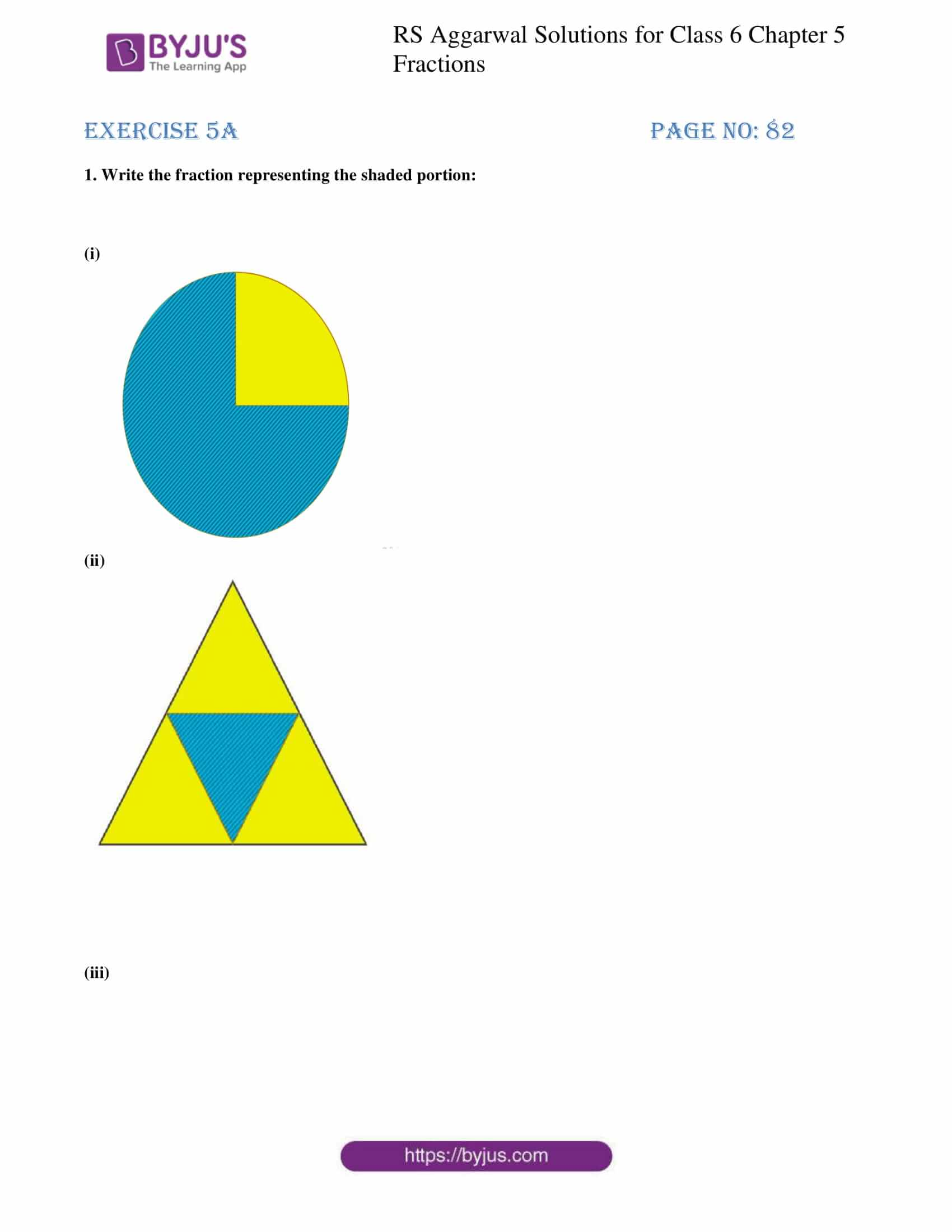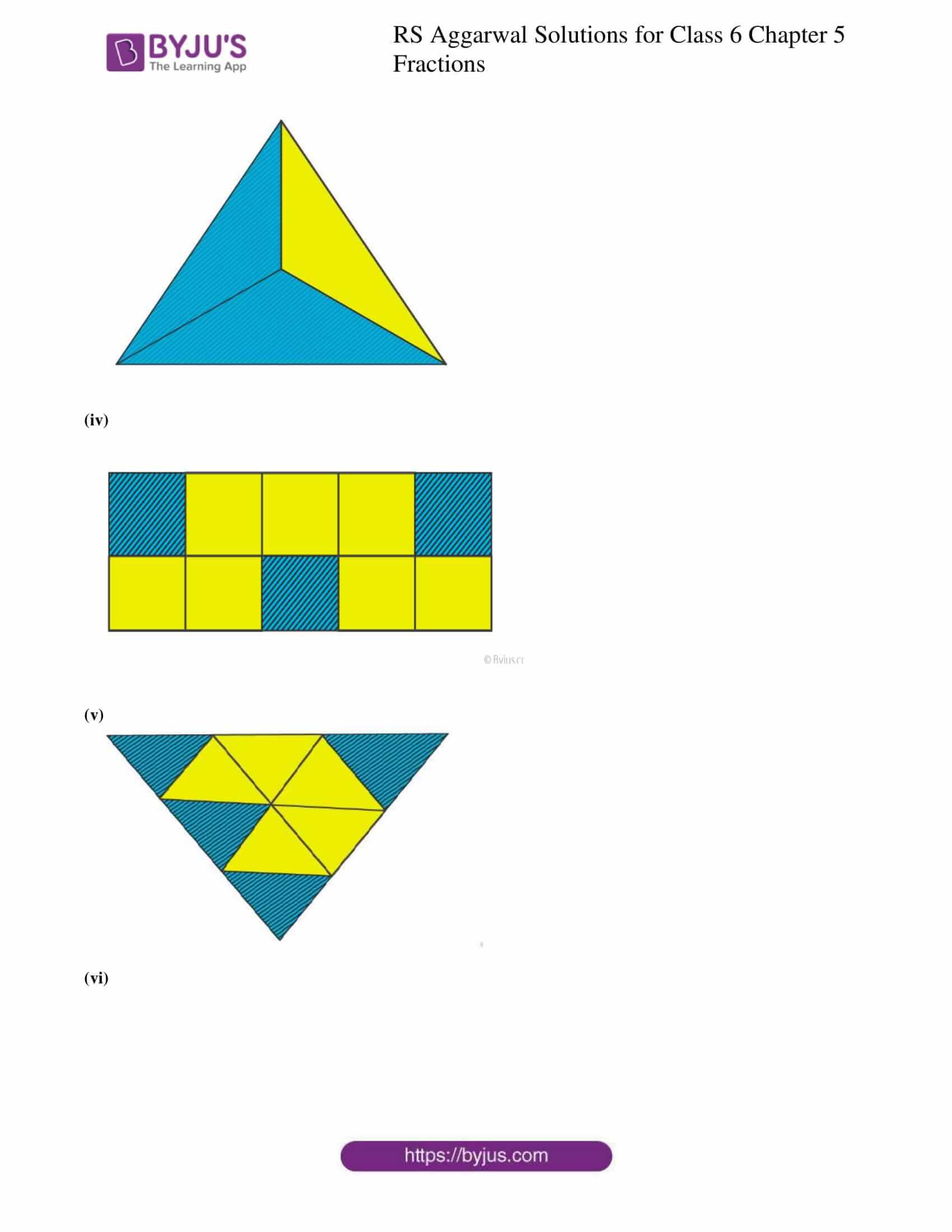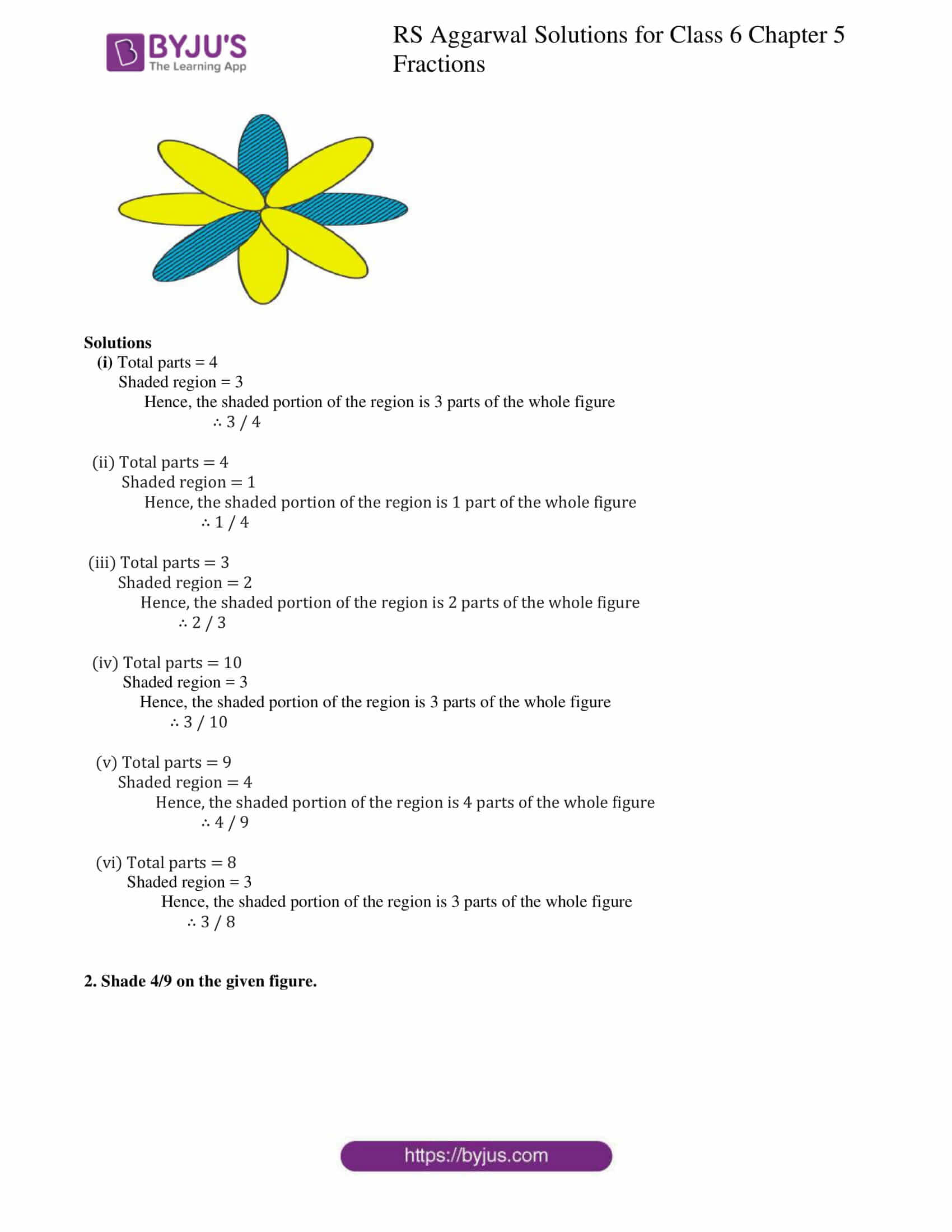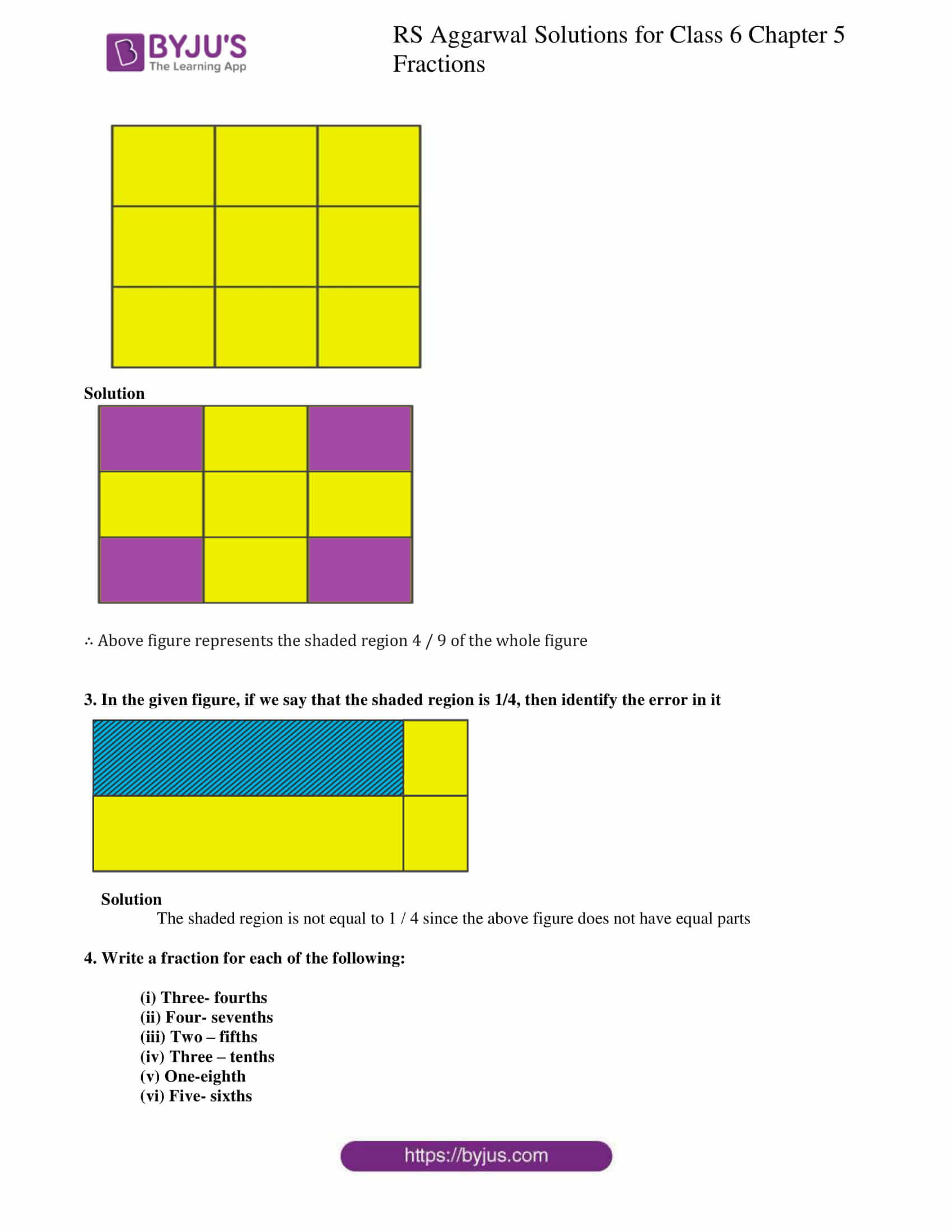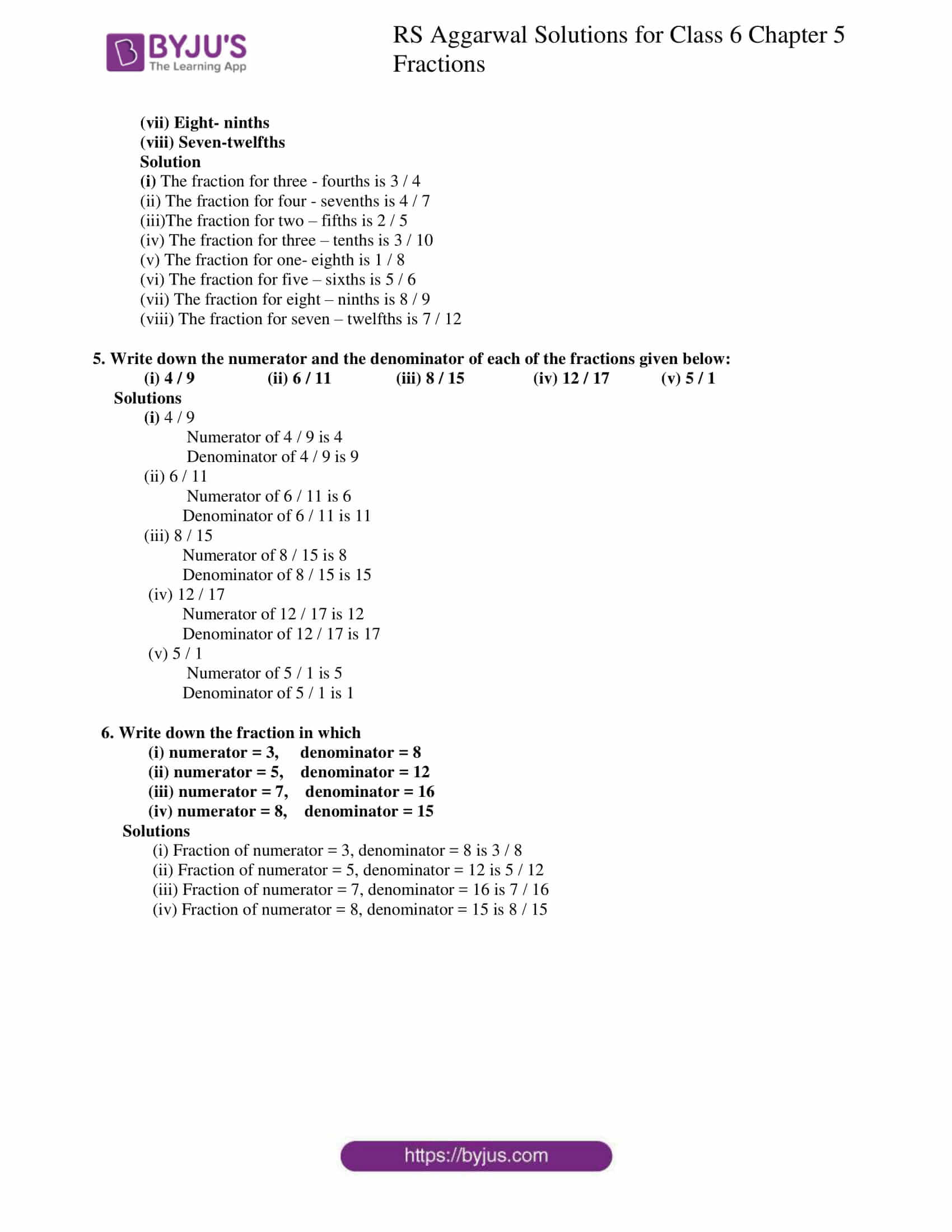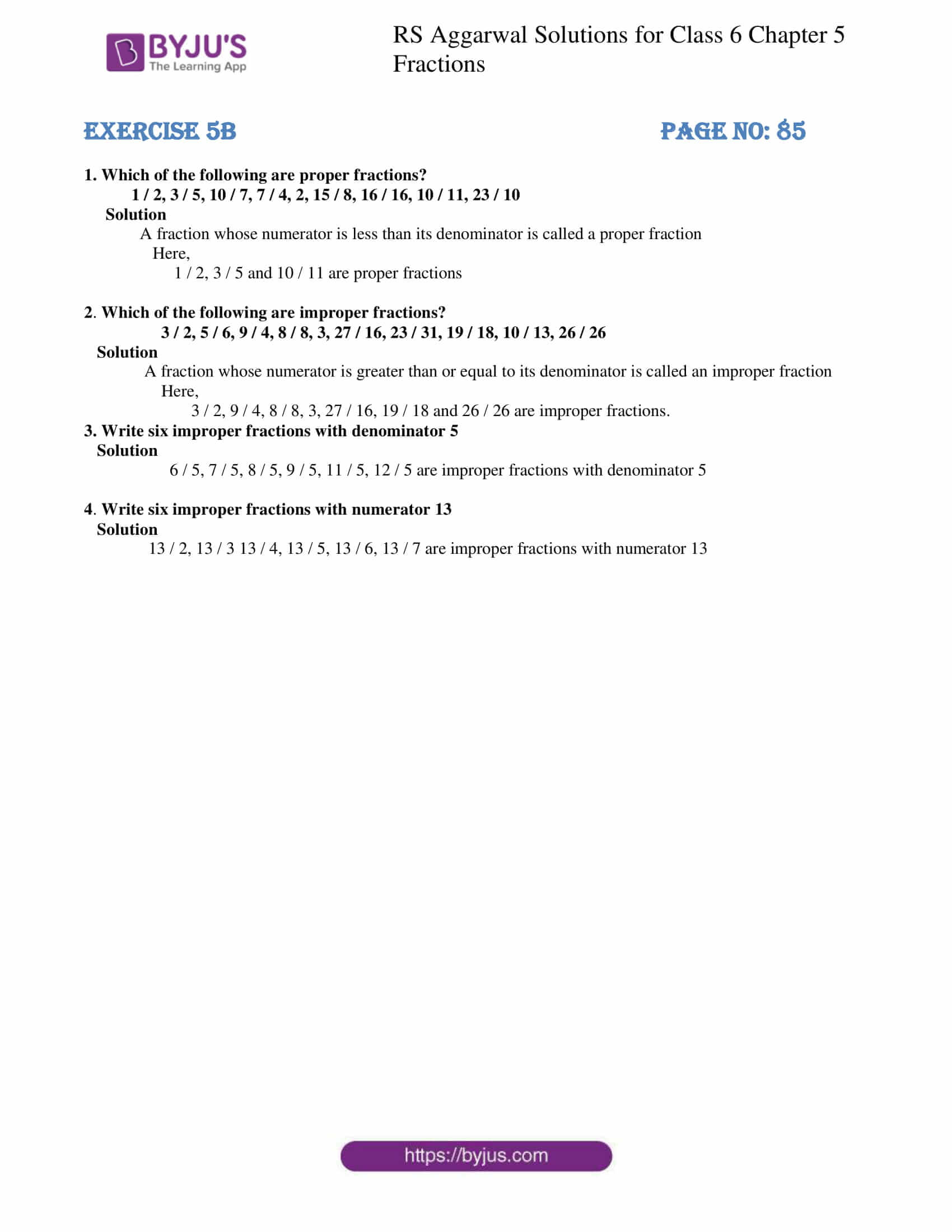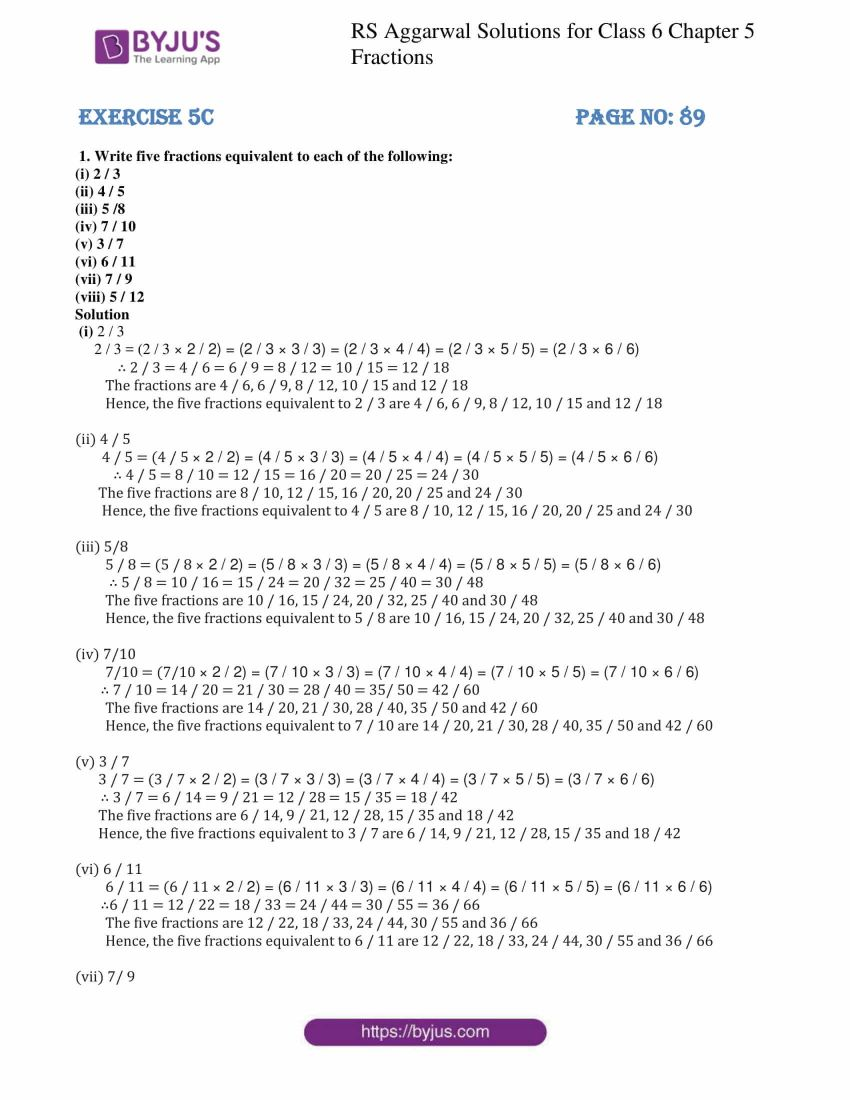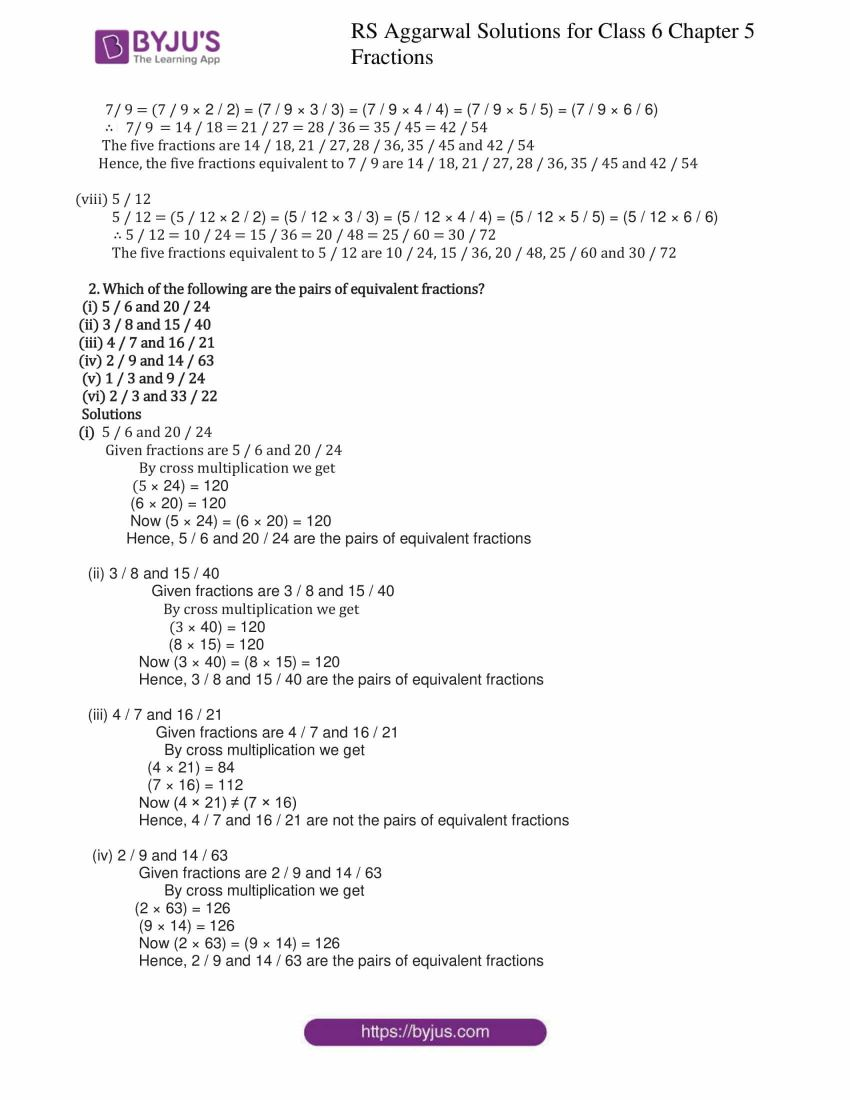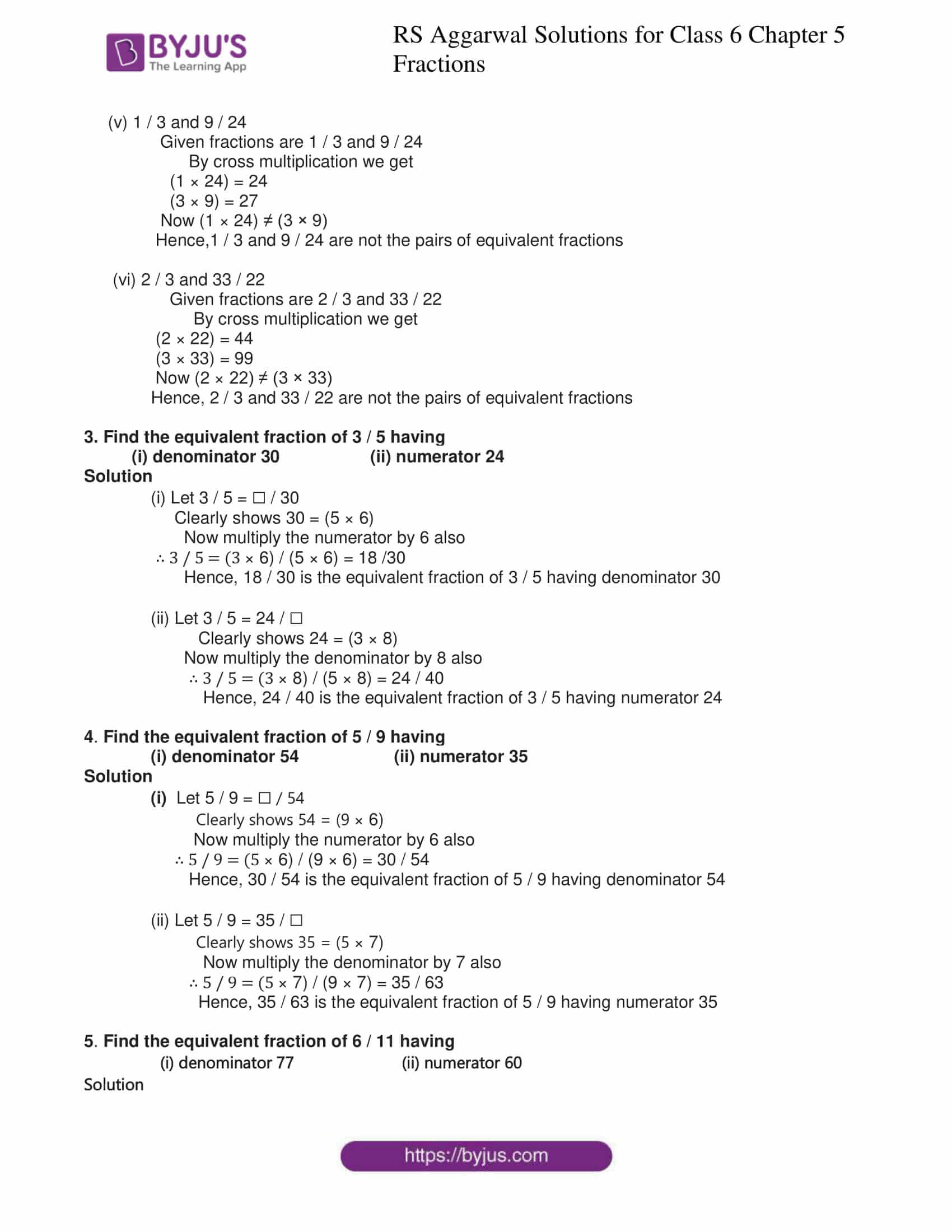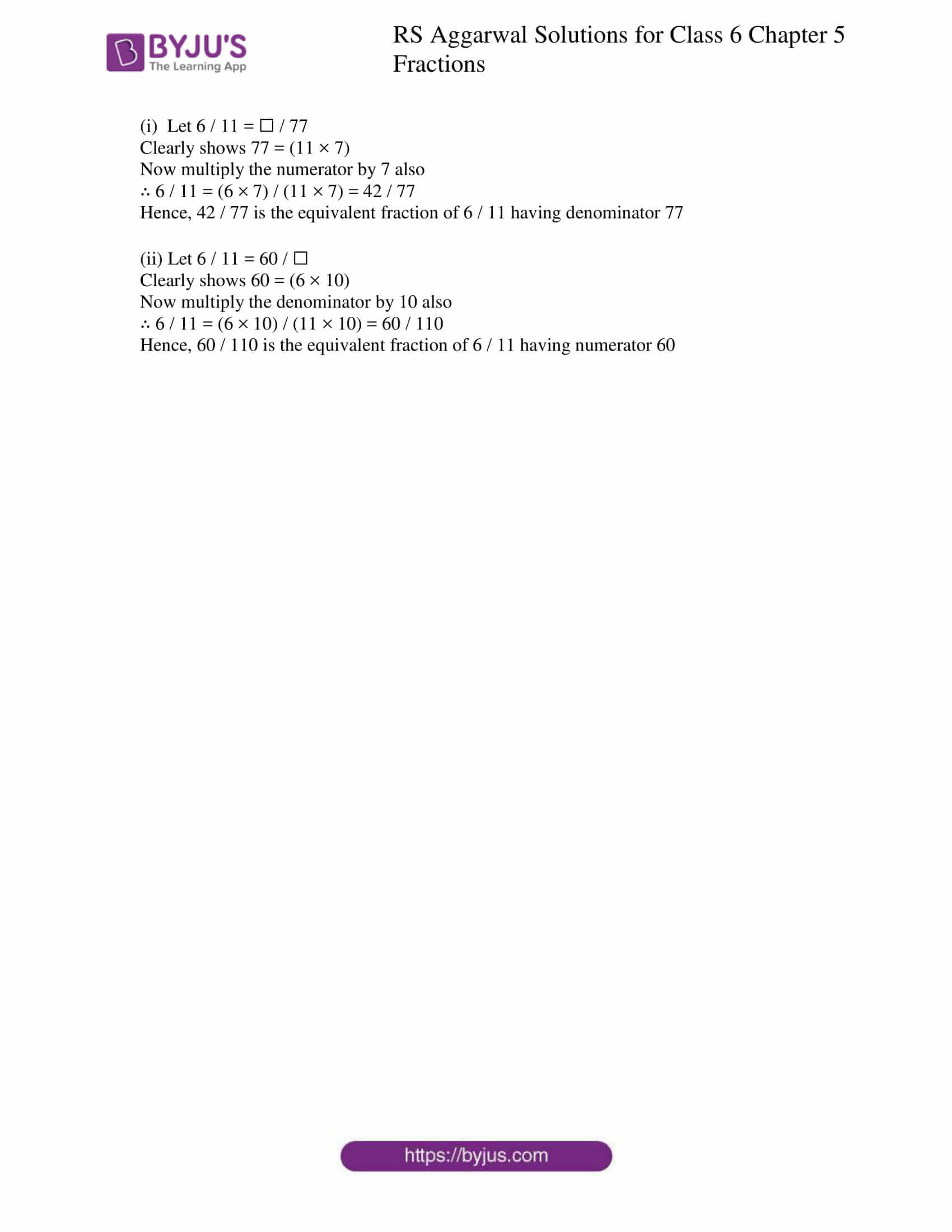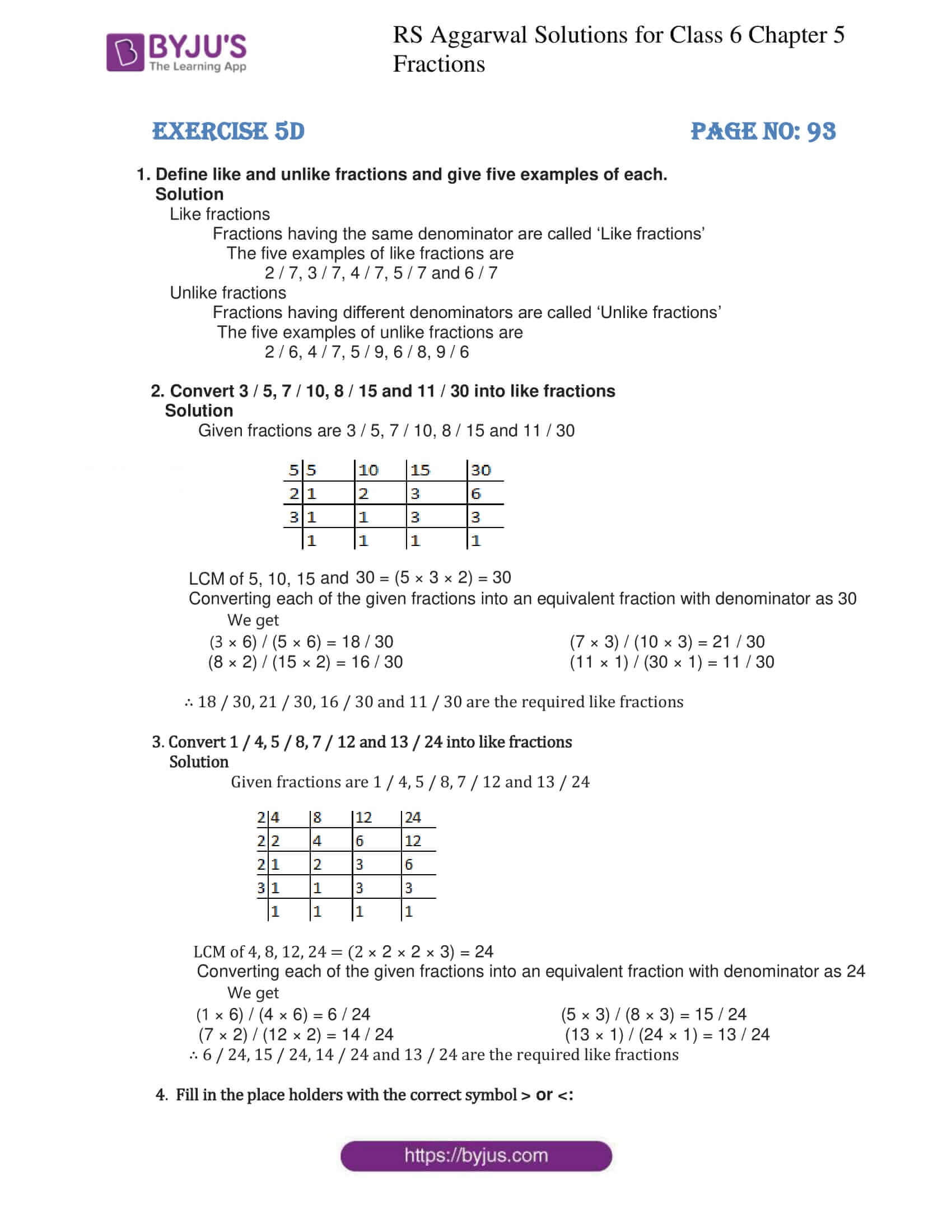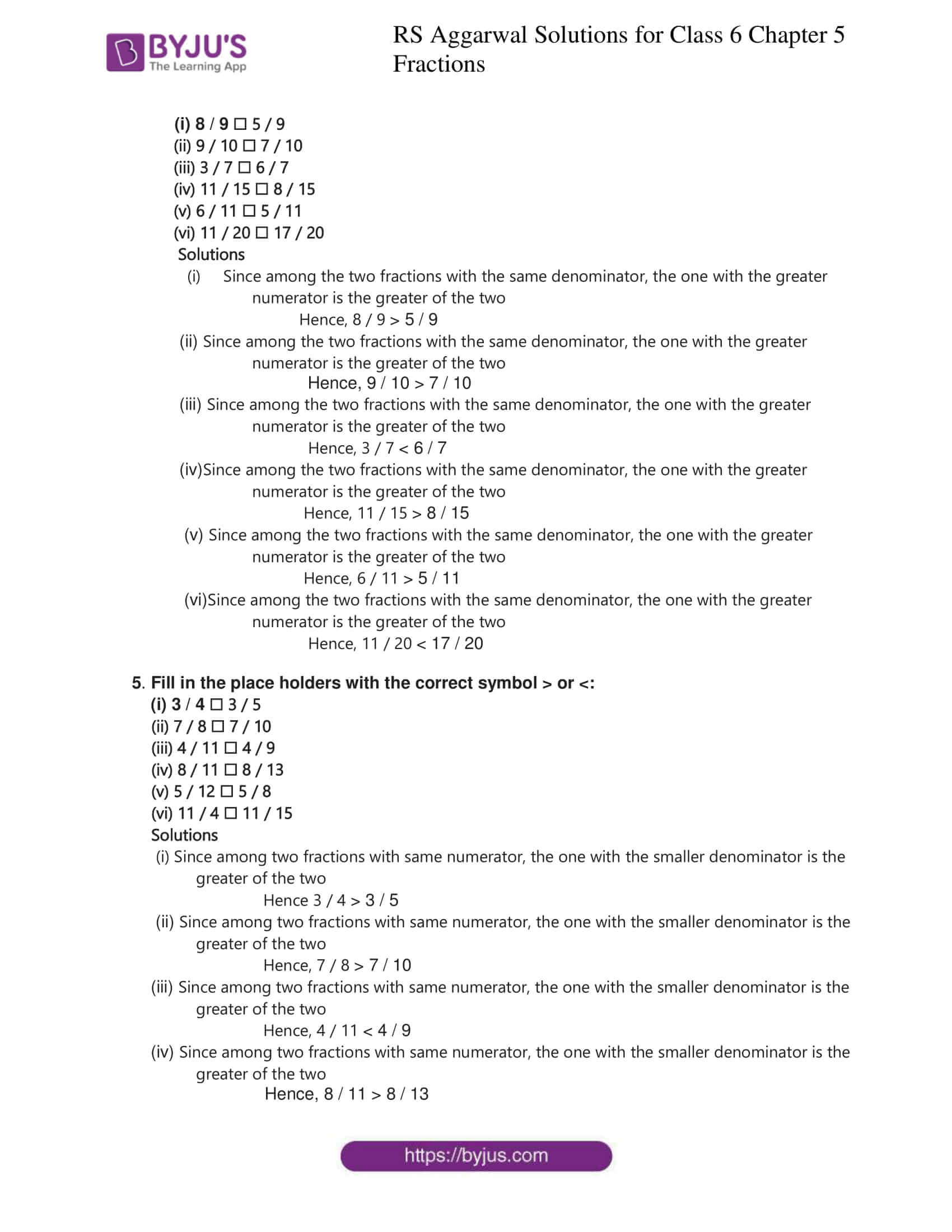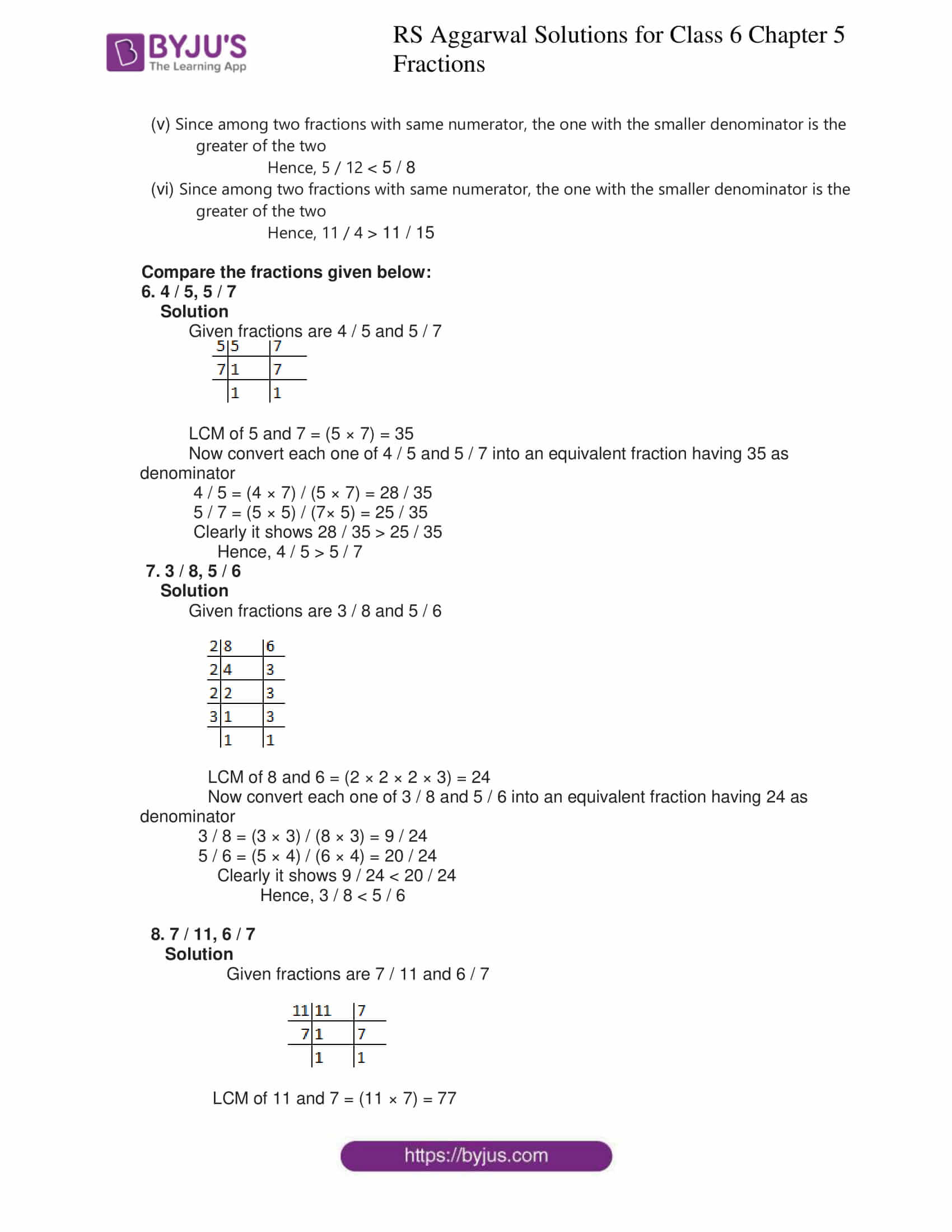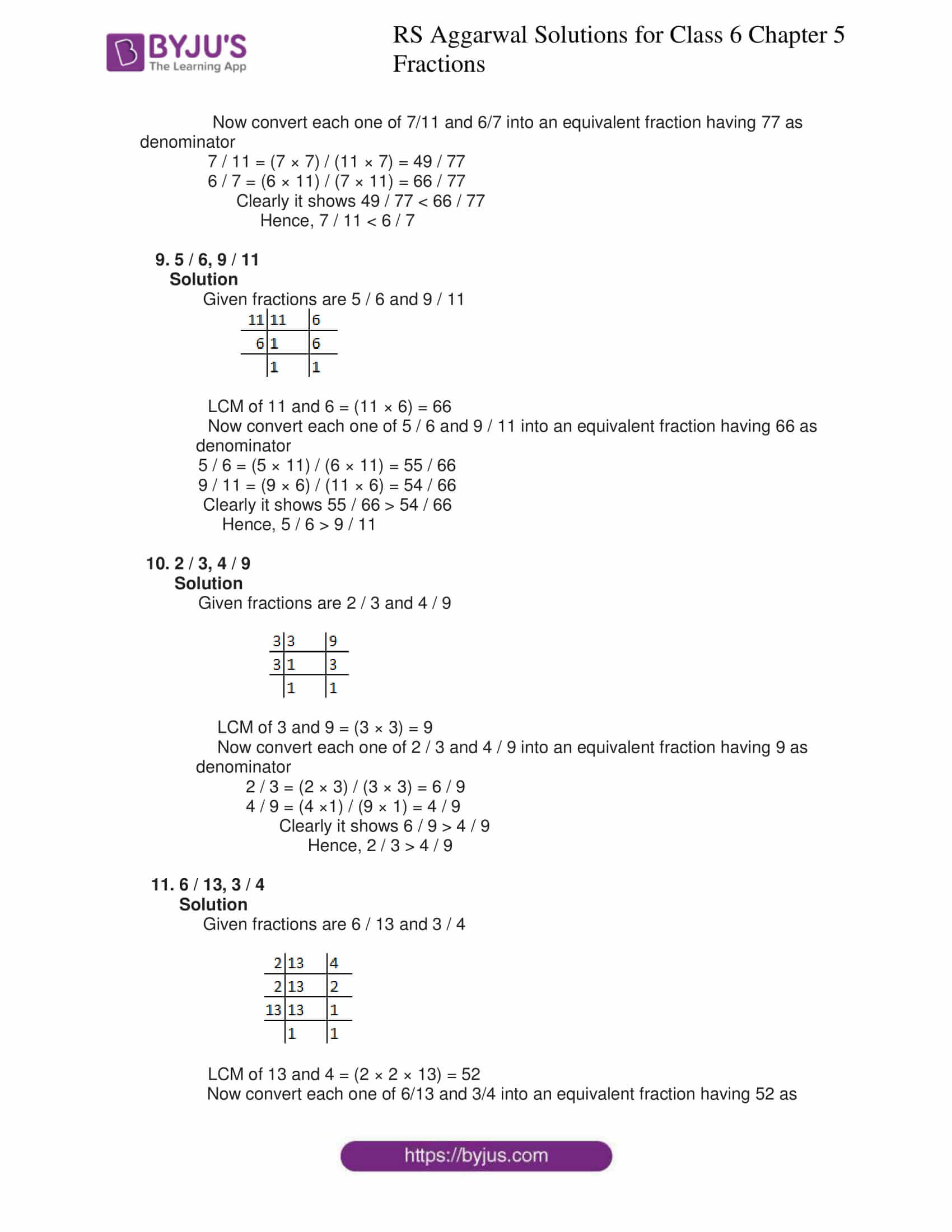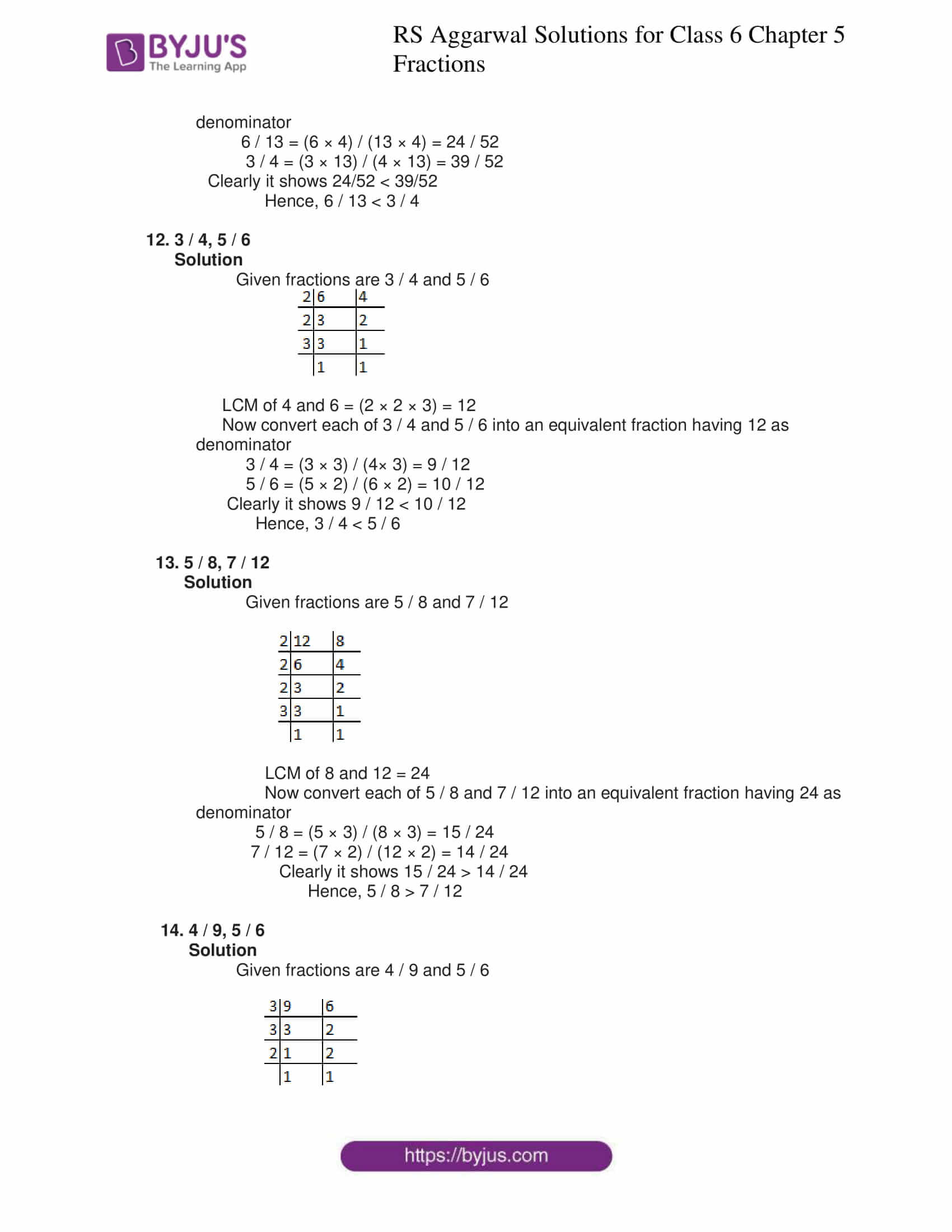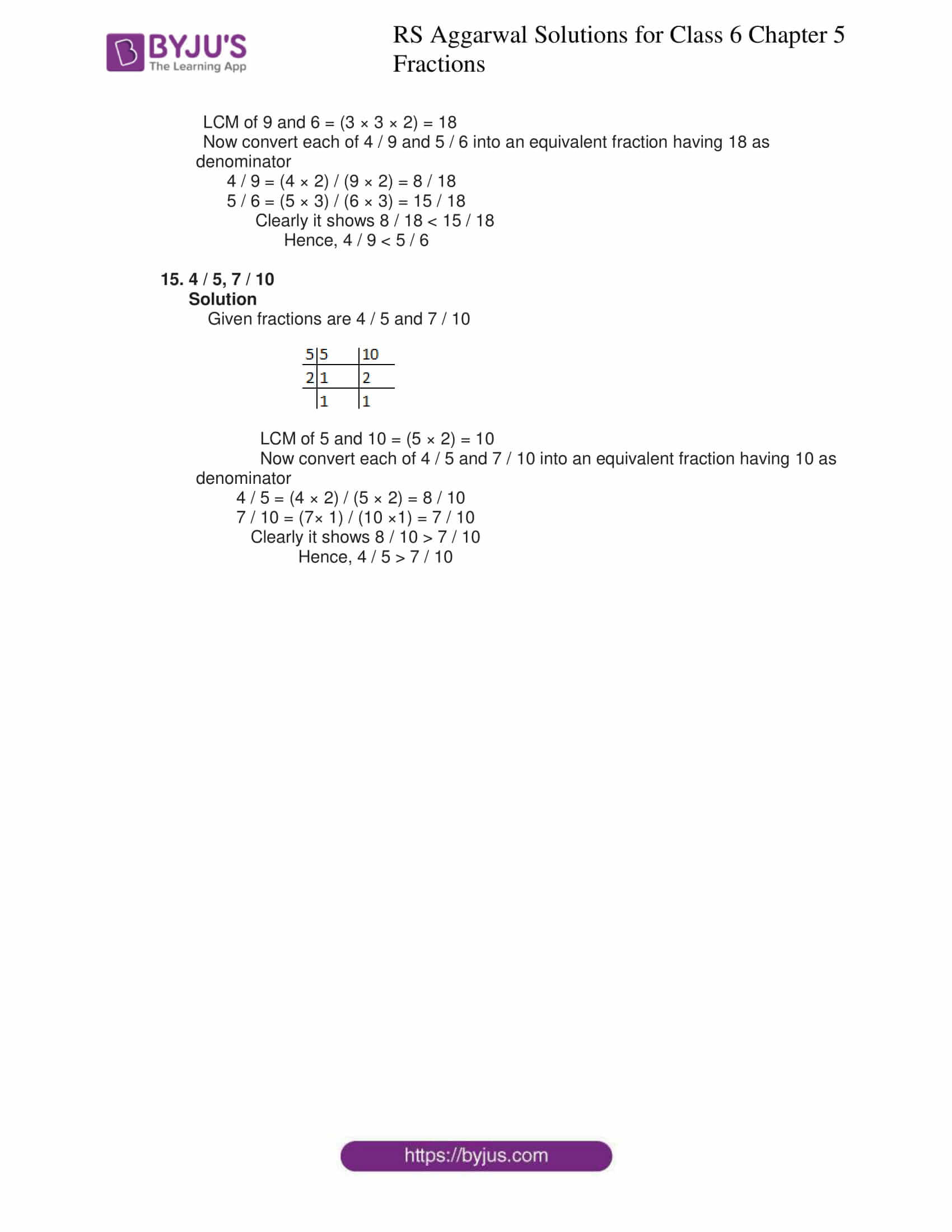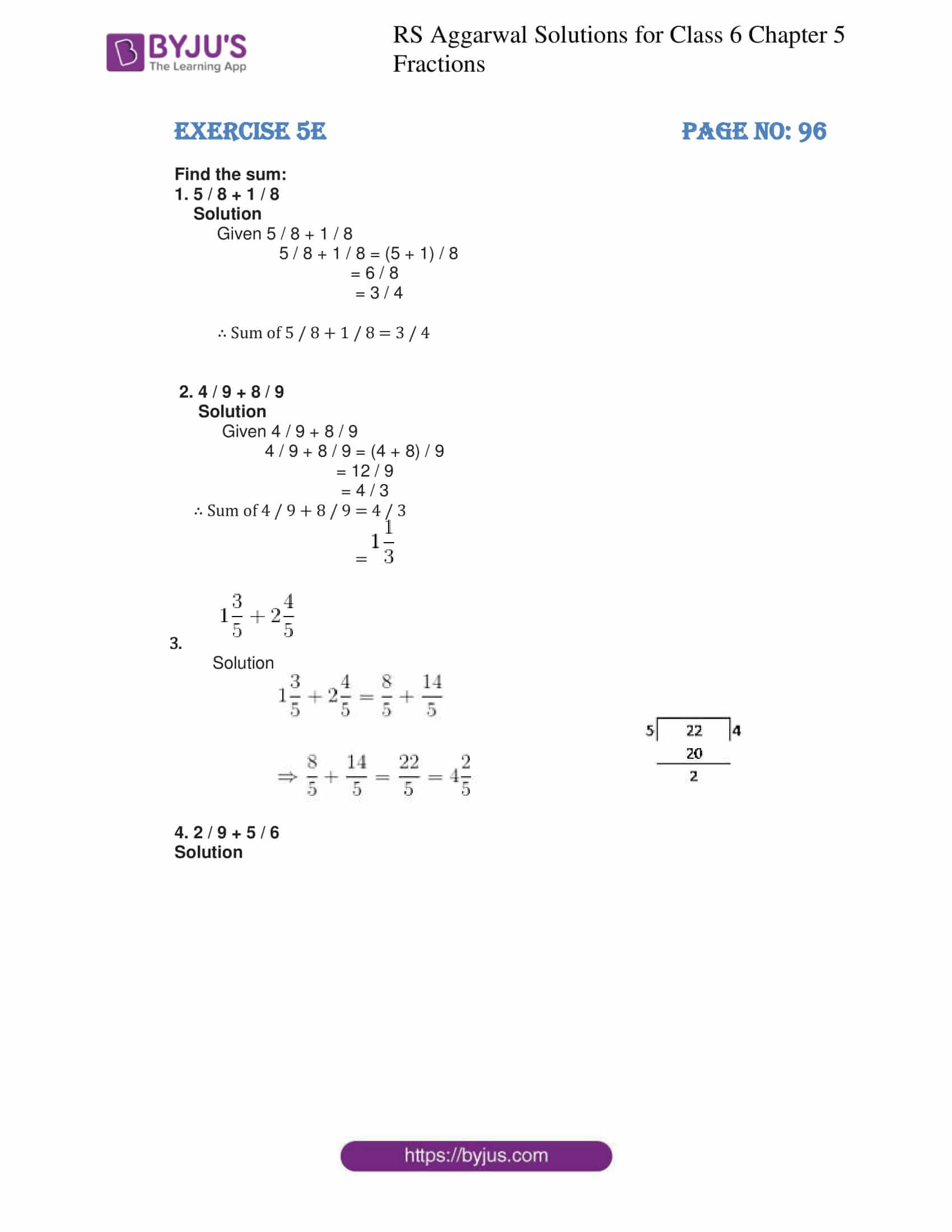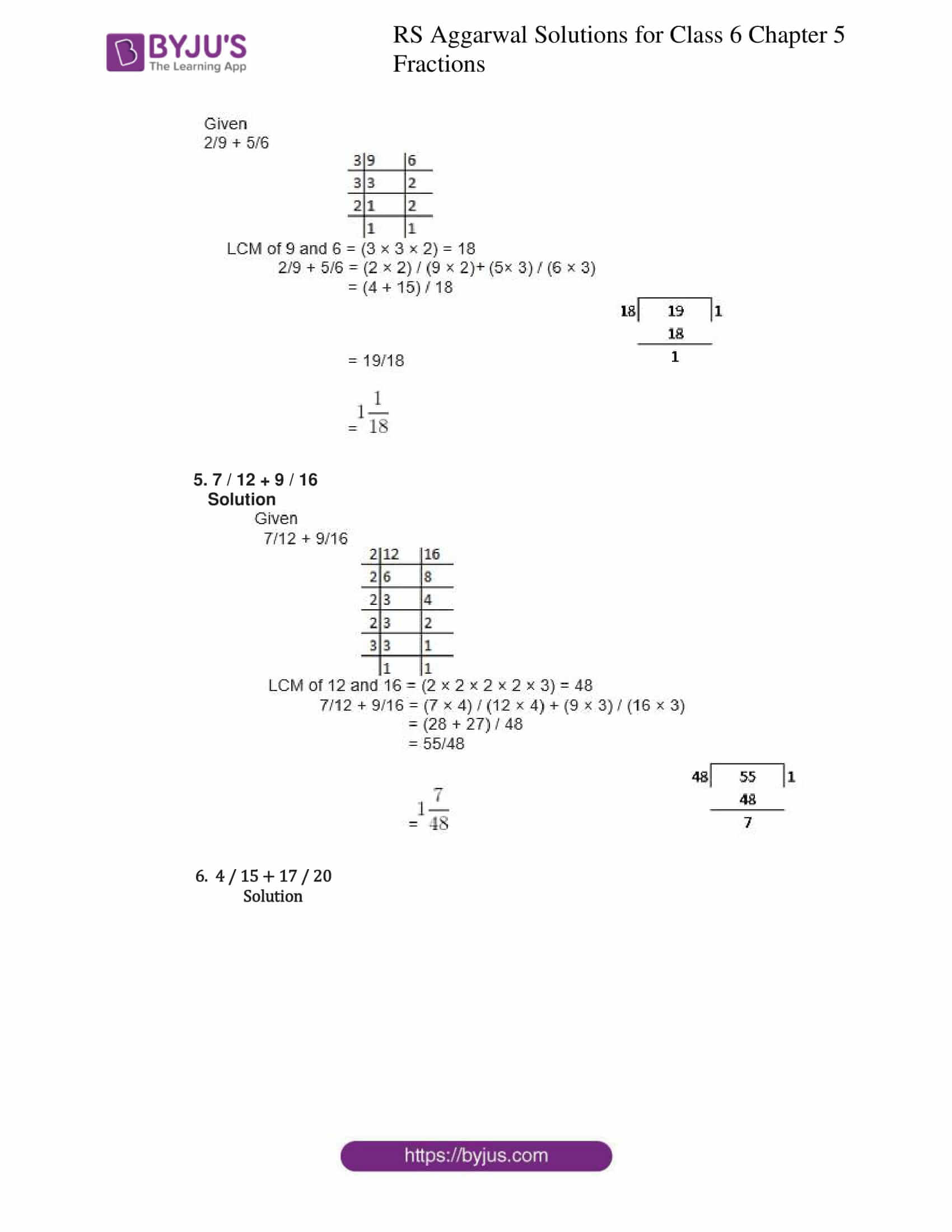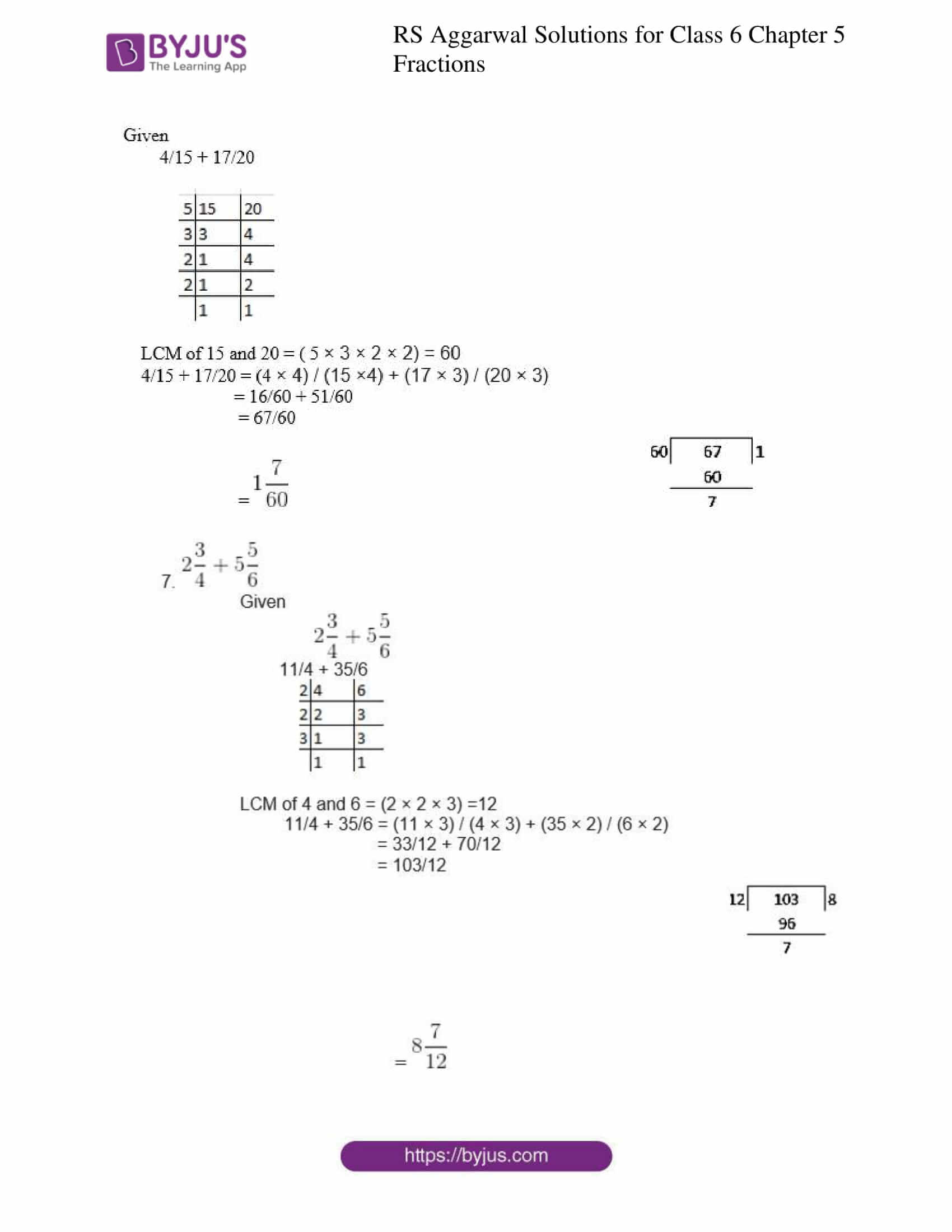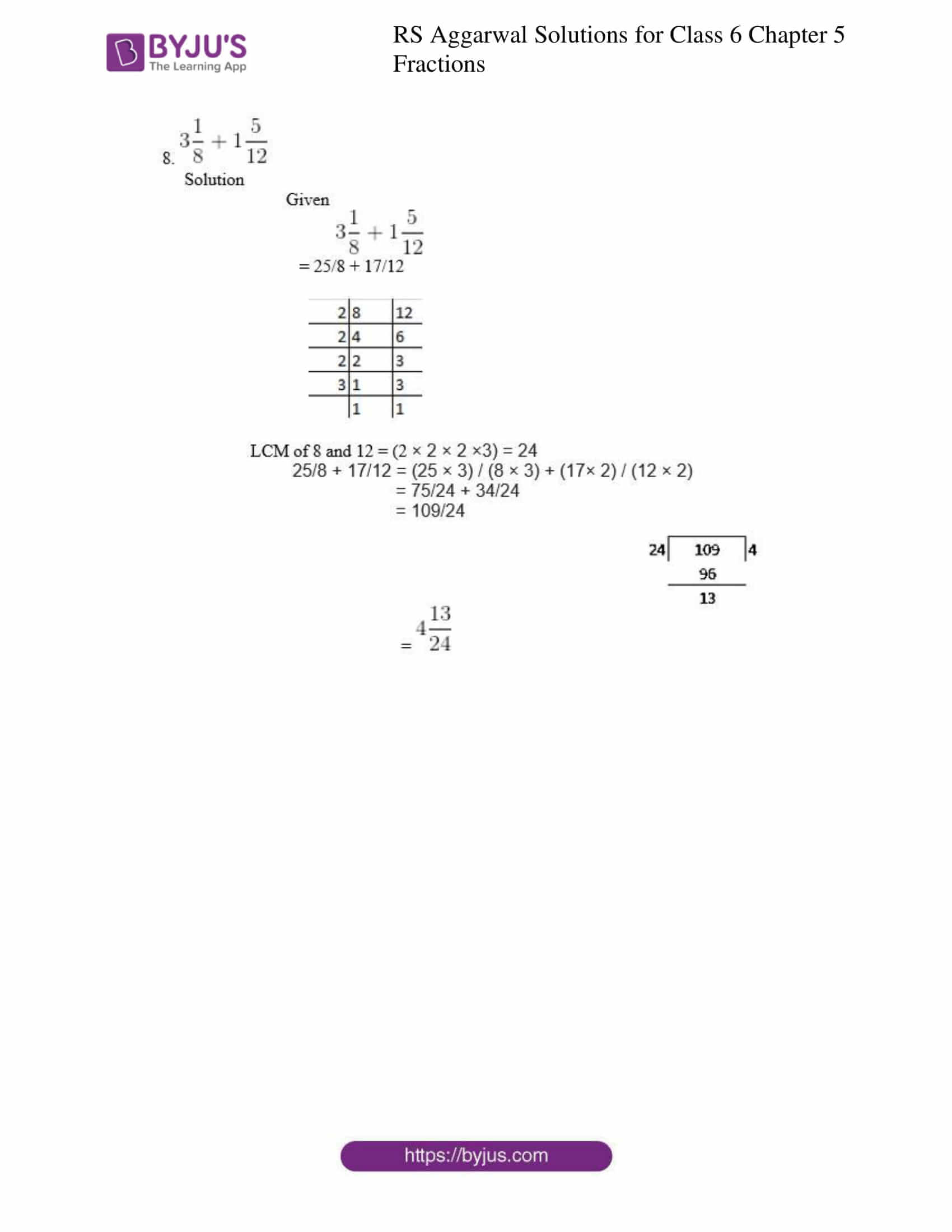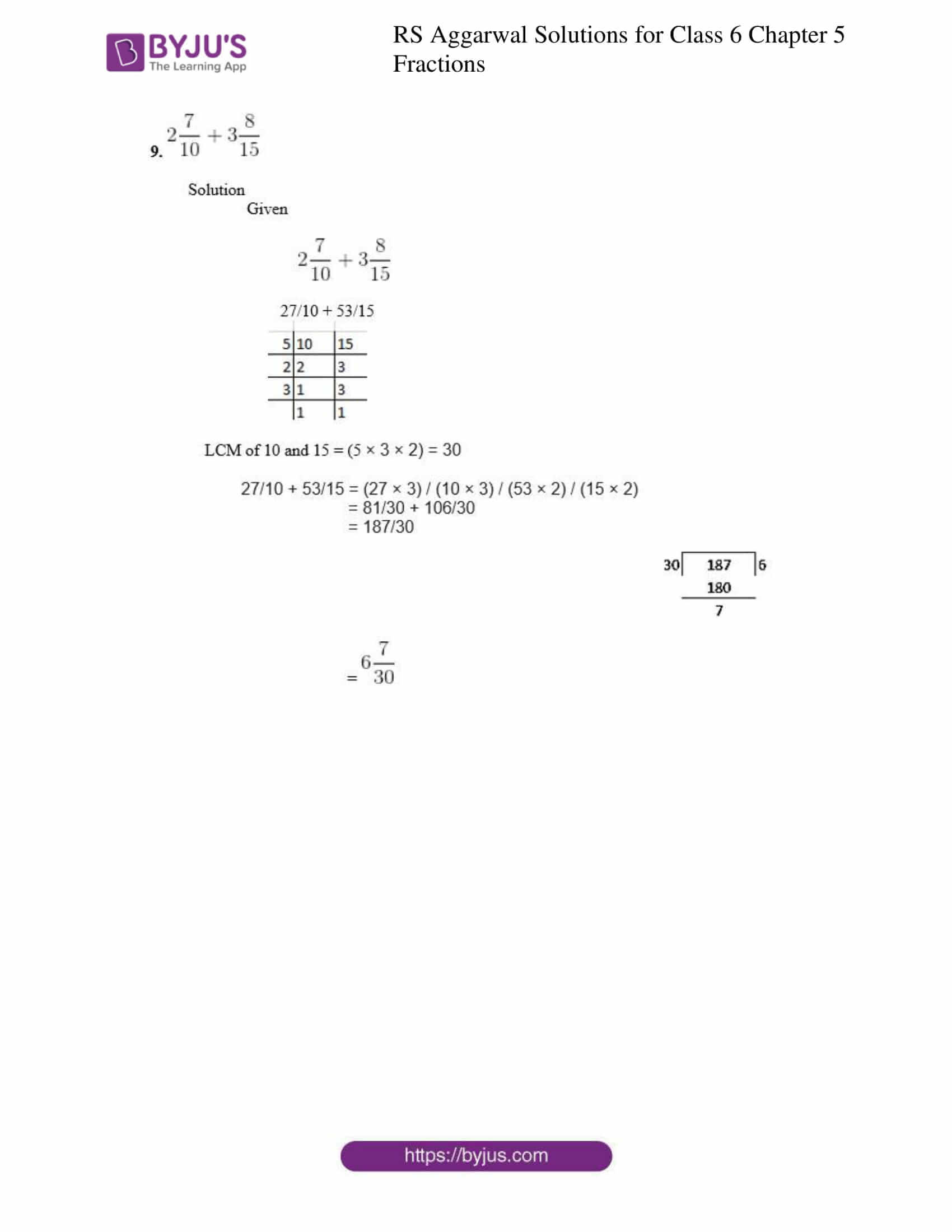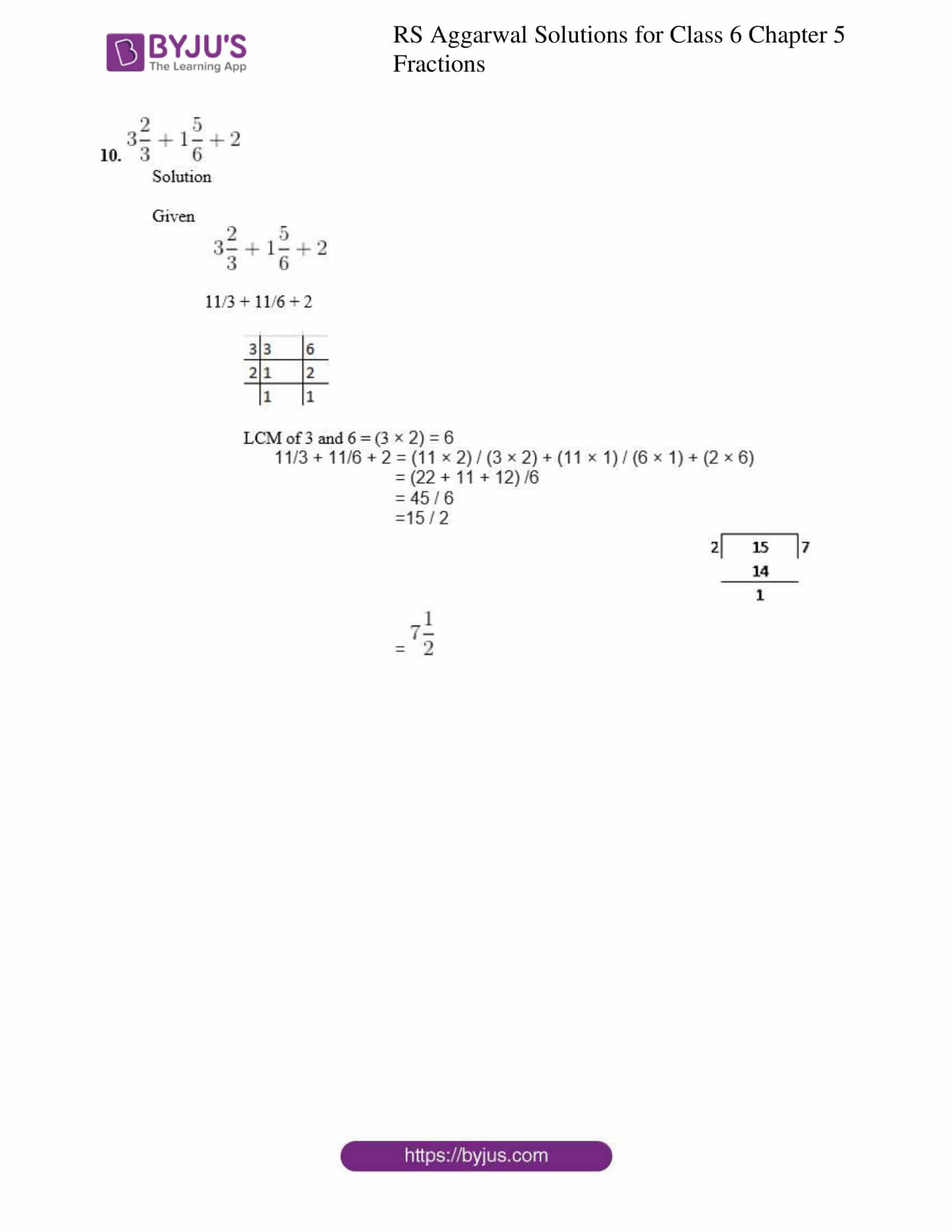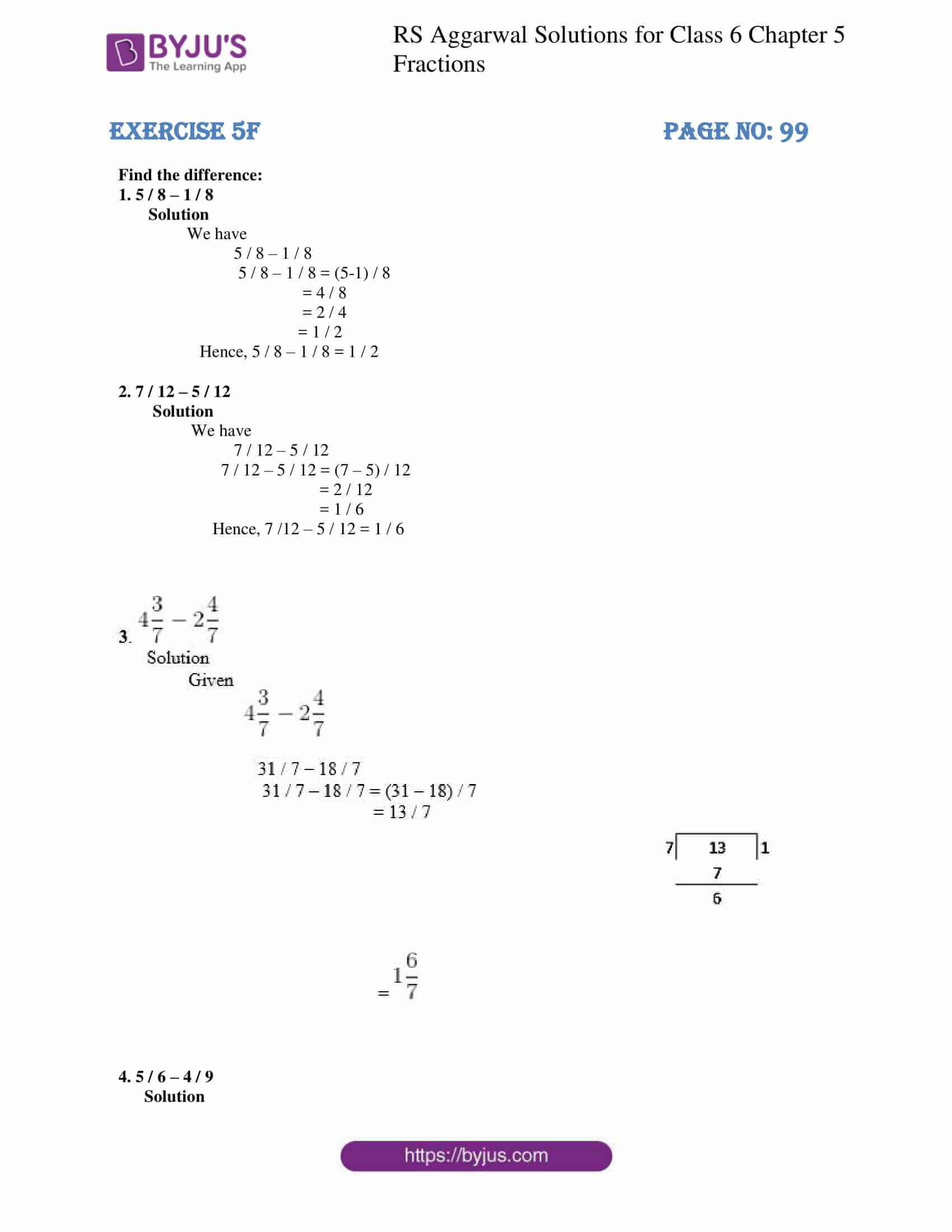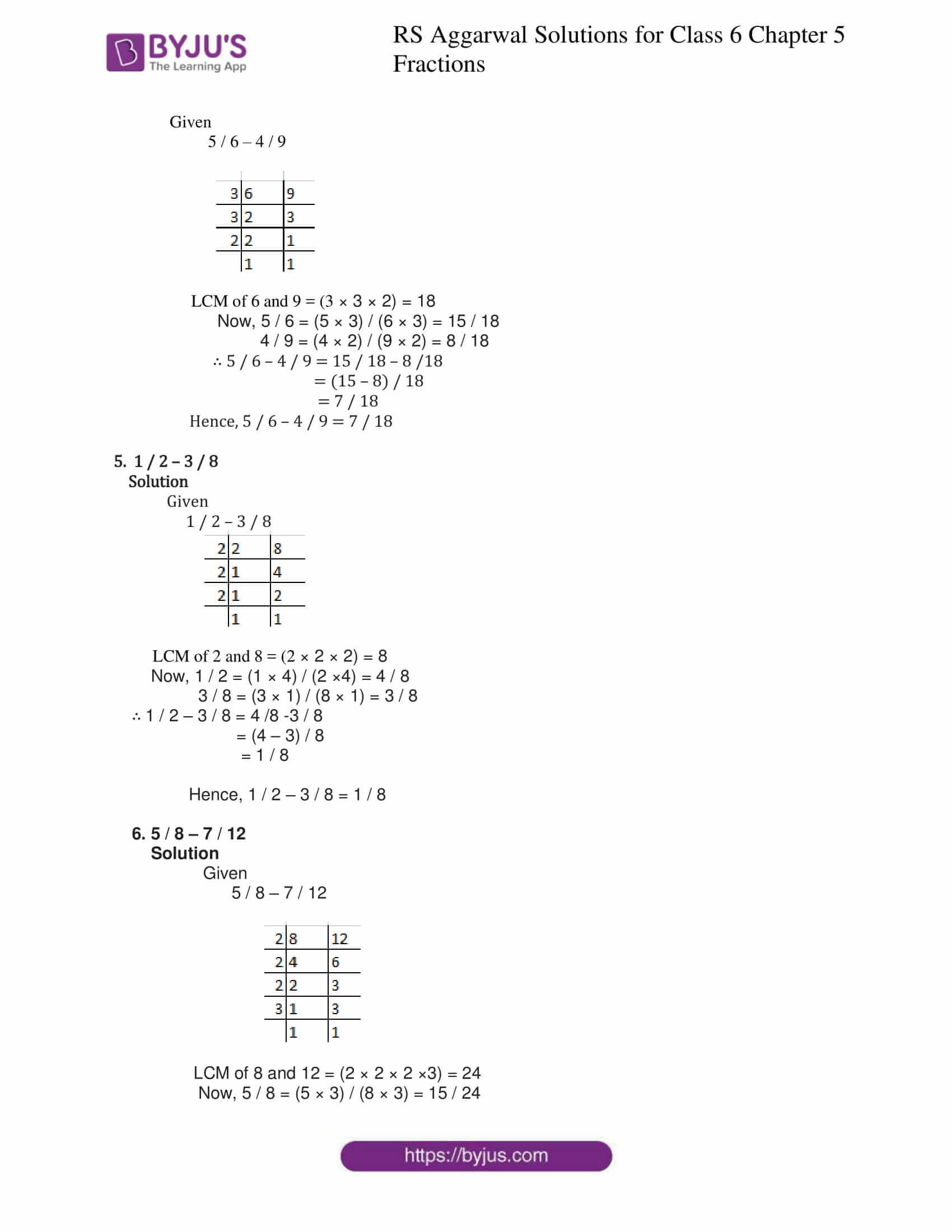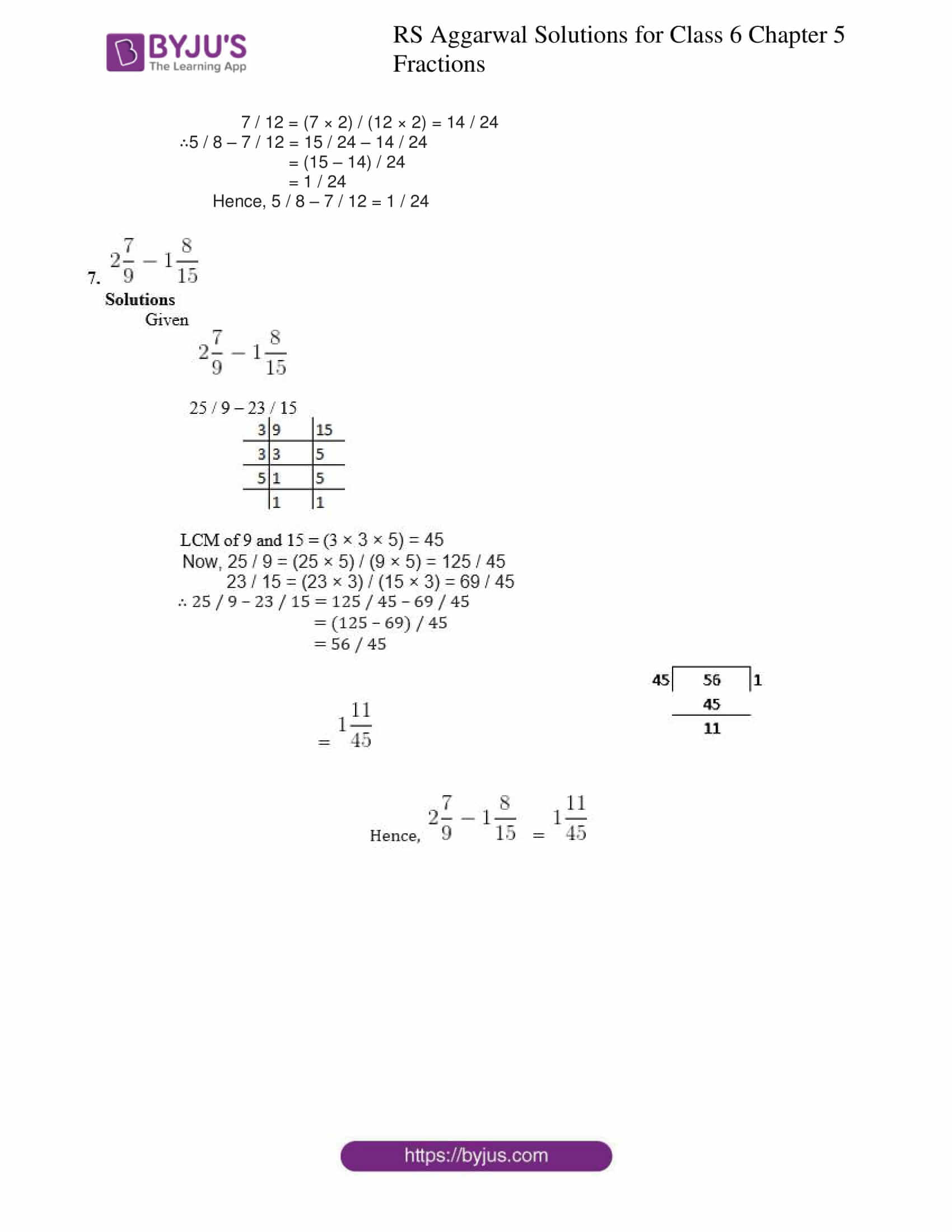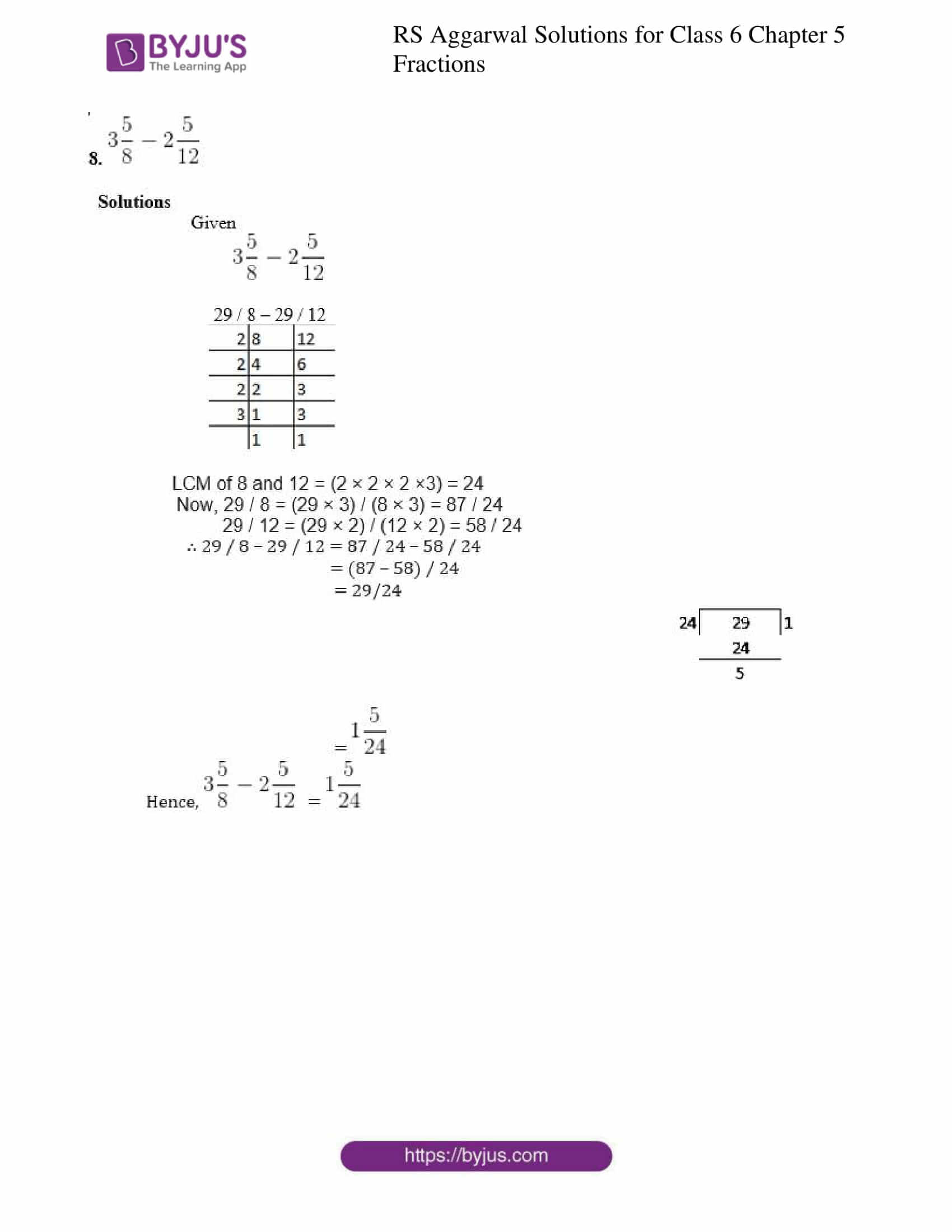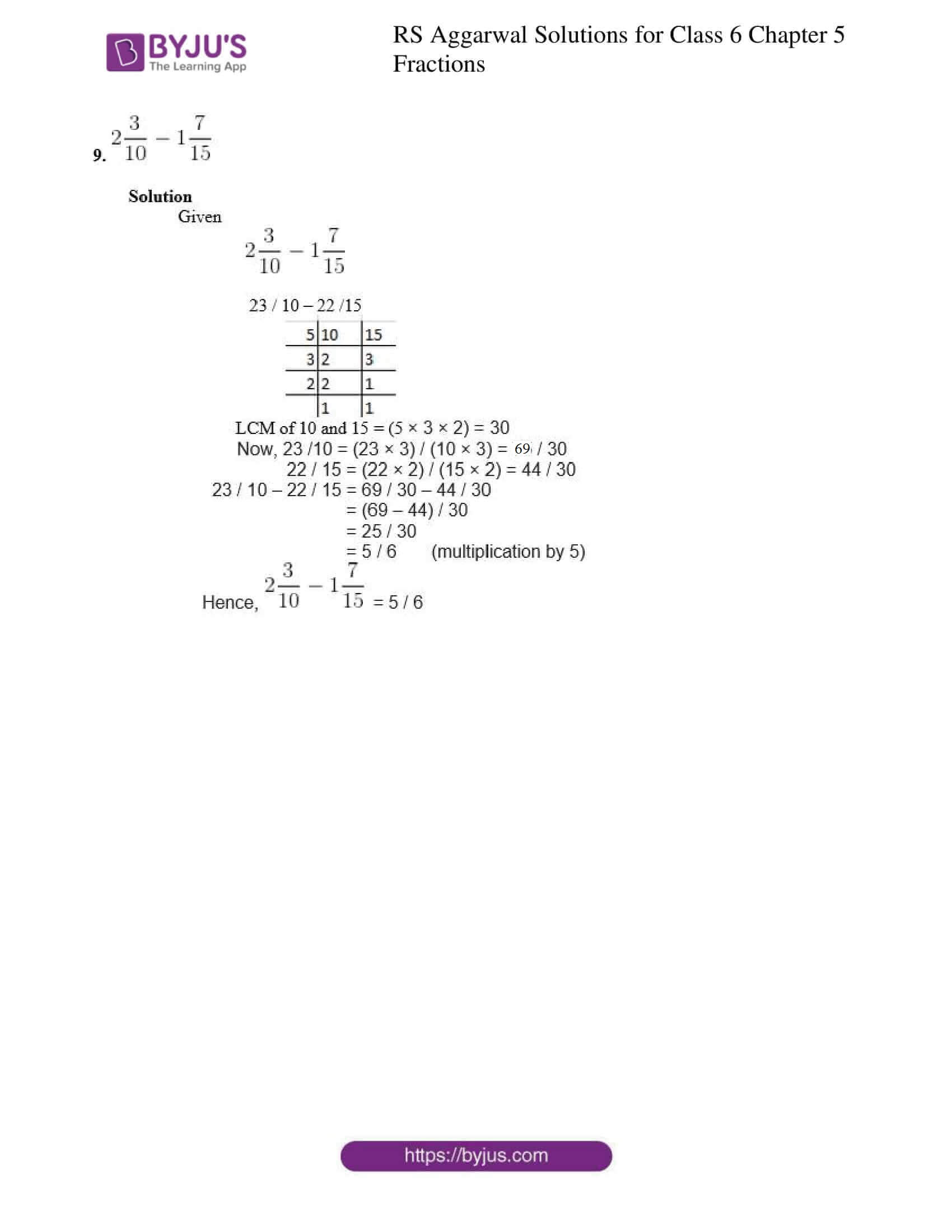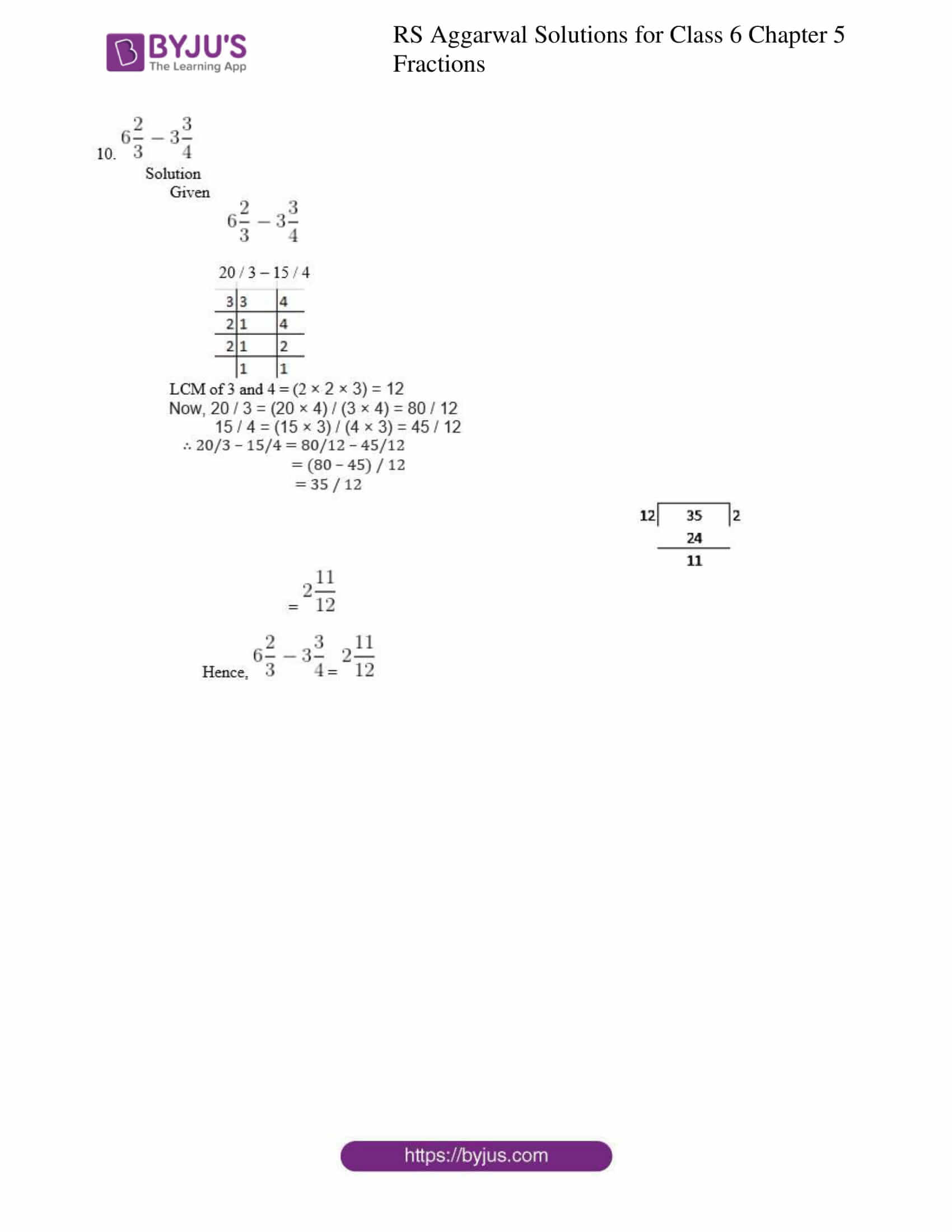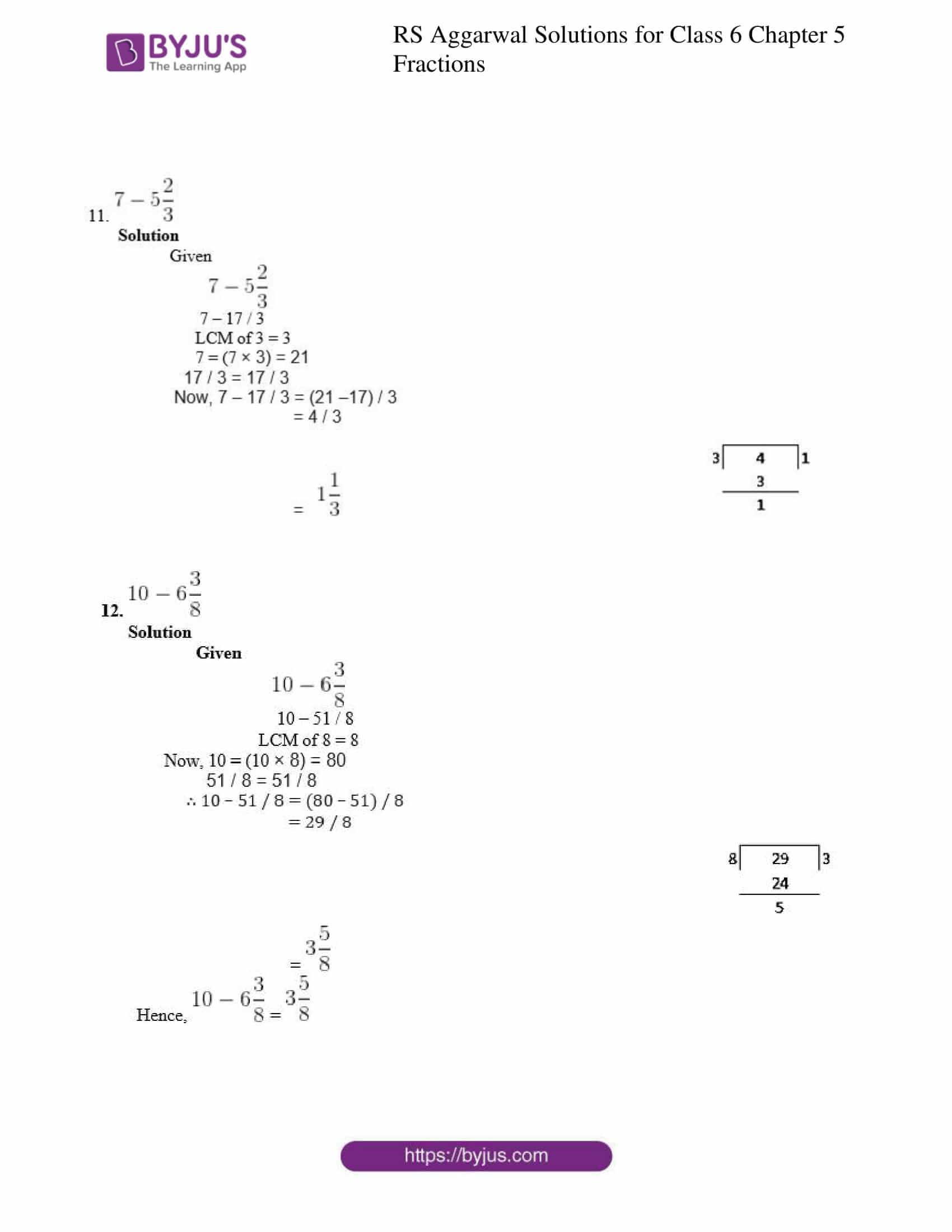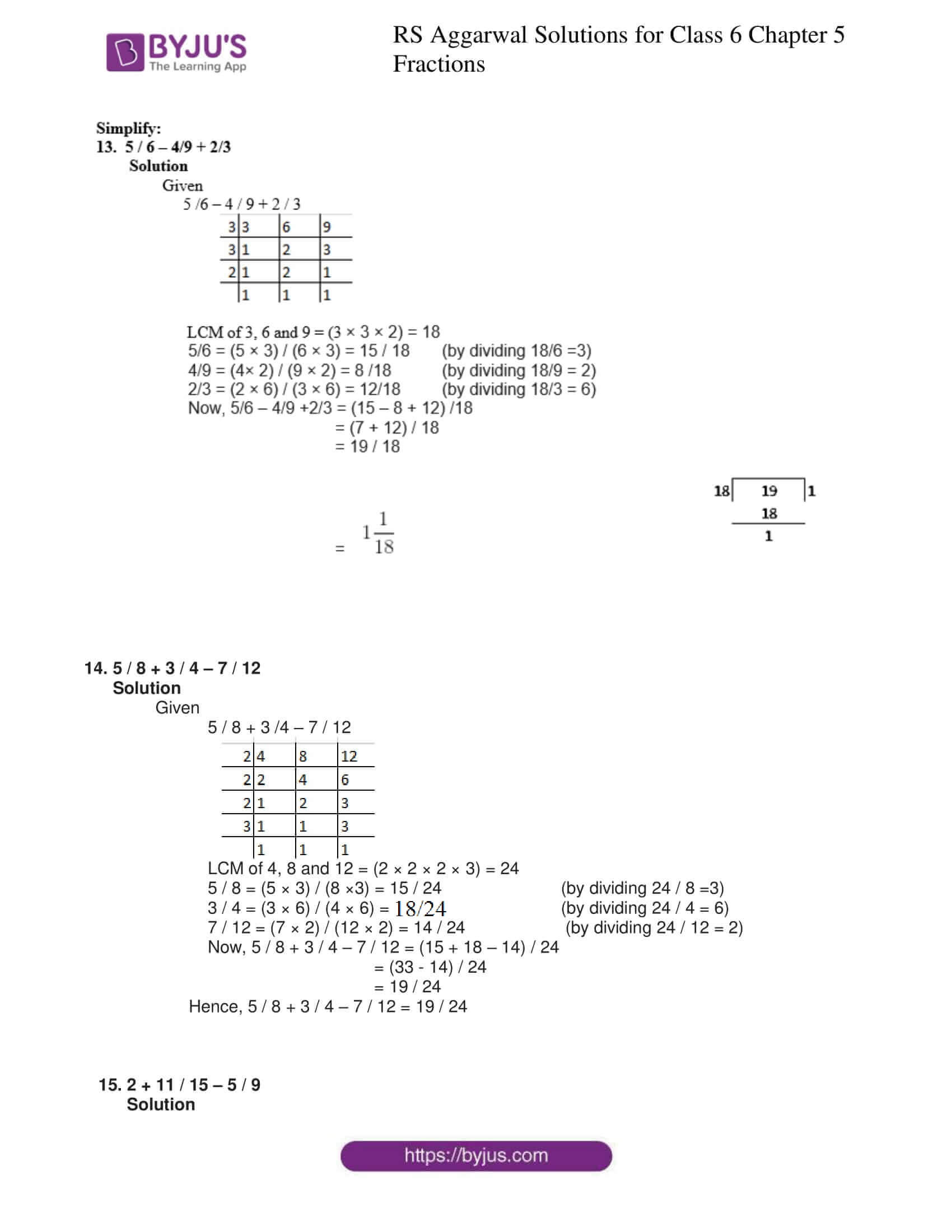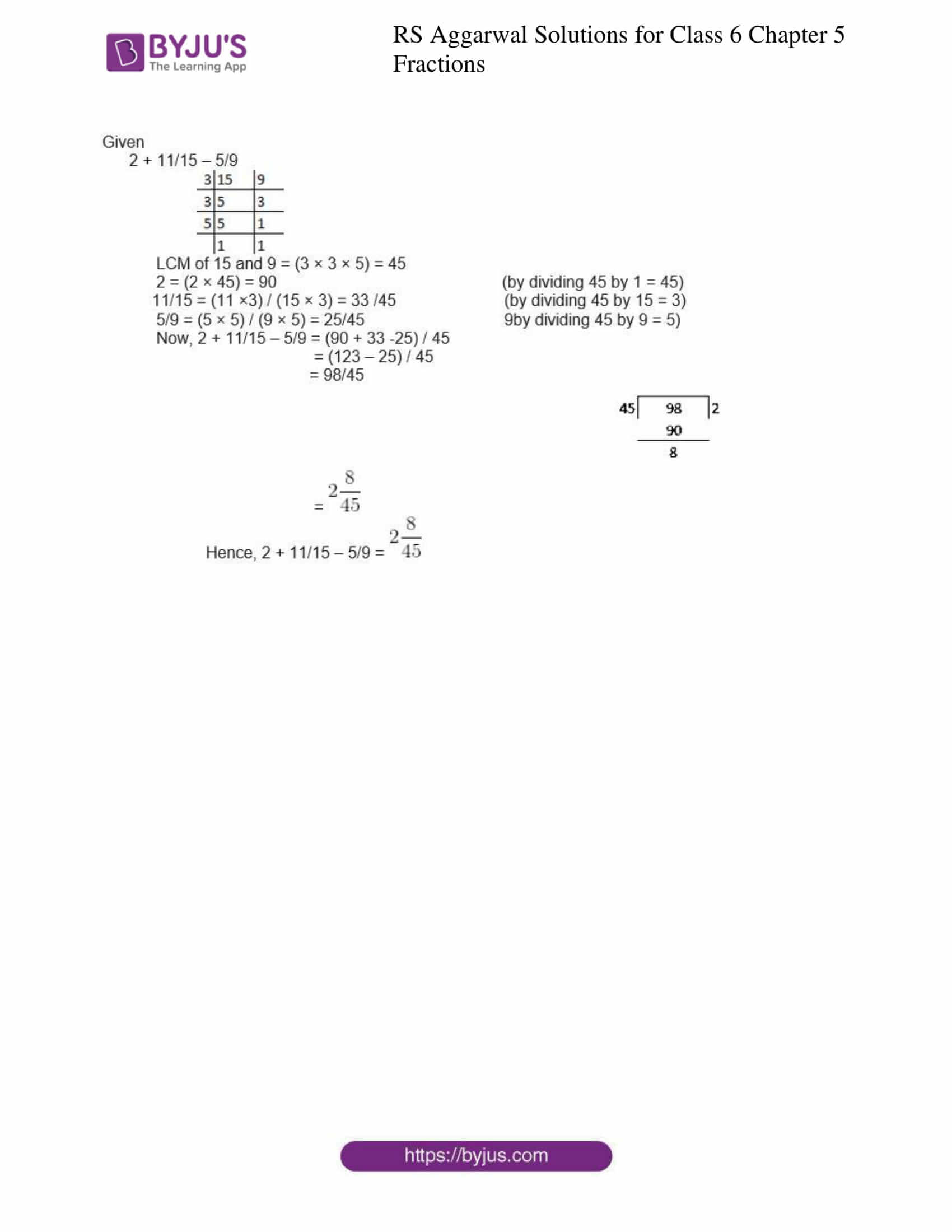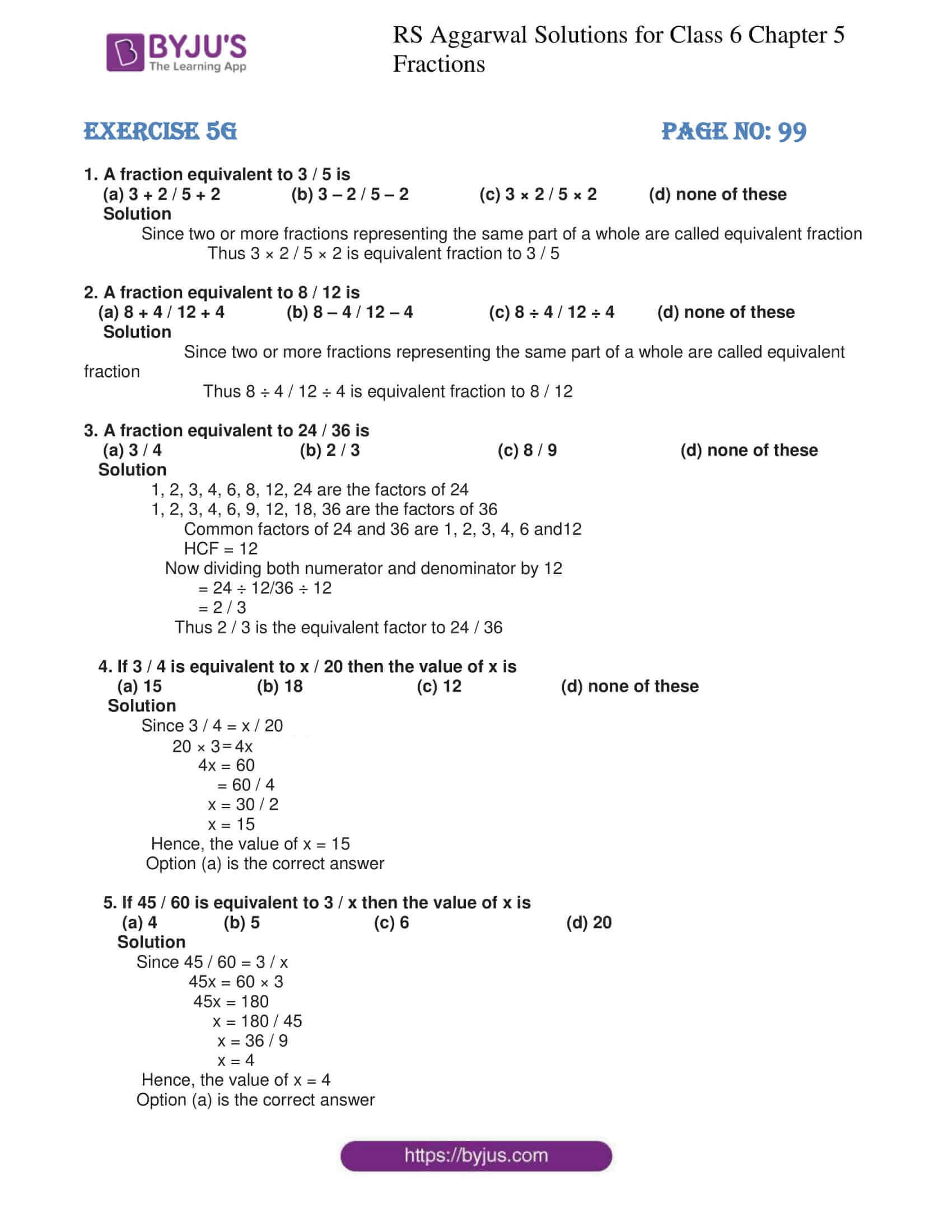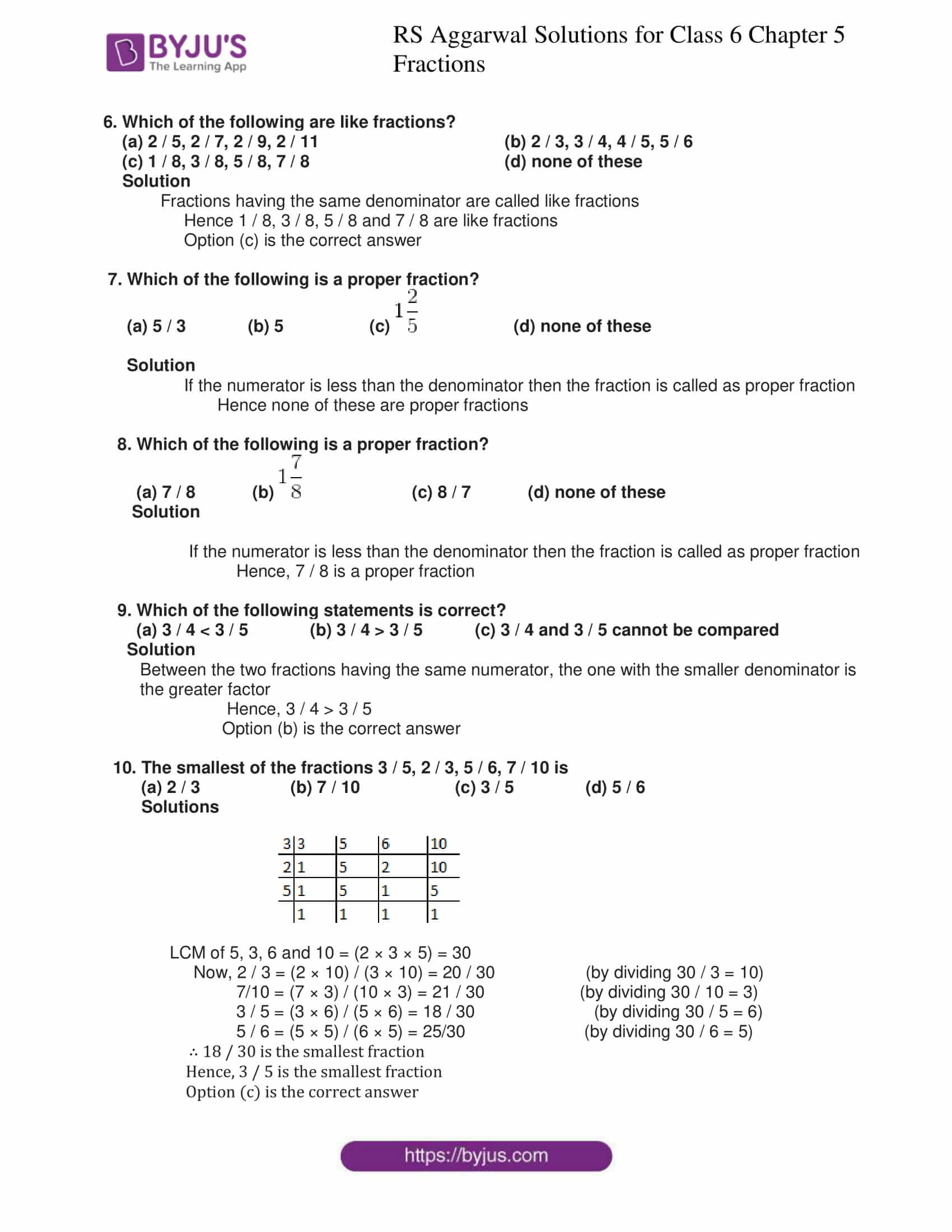## Exercise 5A PAGE NO: 82

1. Write the fraction representing the shaded portion:

(i)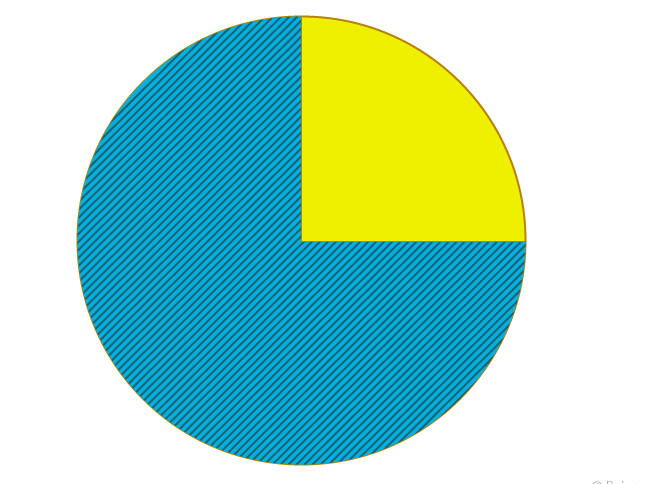(ii)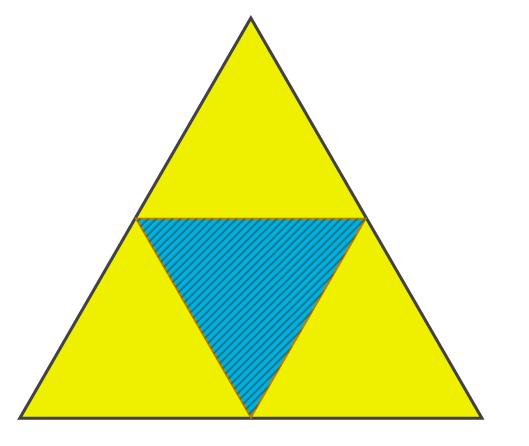(iii)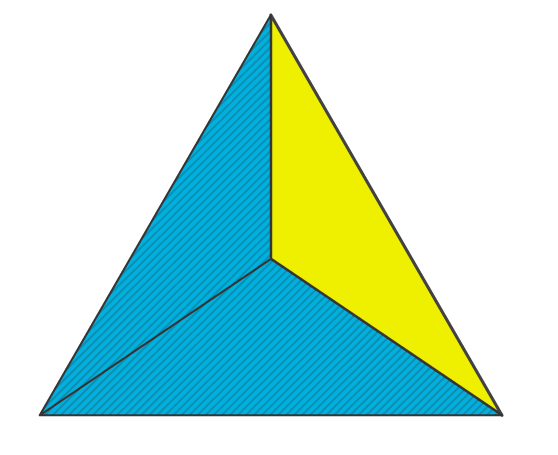(iv)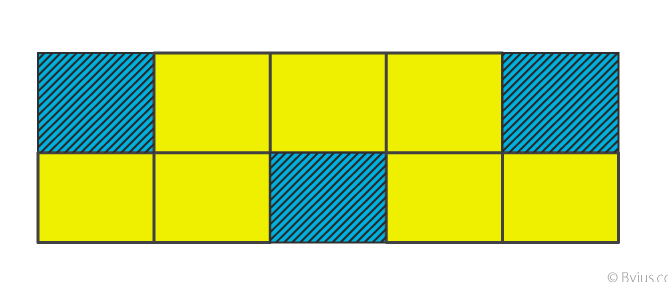(v)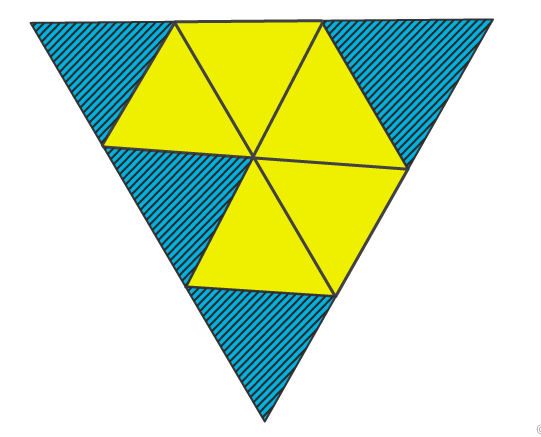(vi)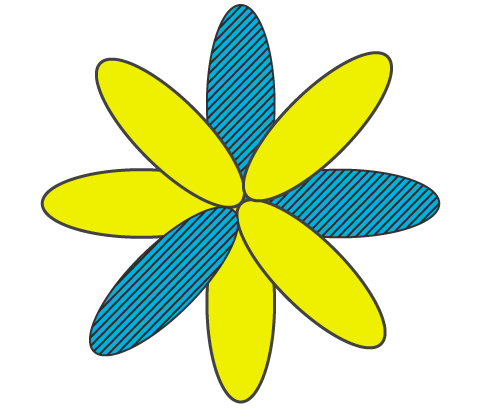Solutions

(i) Total parts = 4

Hence, the shaded portion of the region is 3 parts of the whole figure

∴ 3 / 4

(ii) Total parts = 4

Hence, the shaded portion of the region is 1 part of the whole figure

∴ 1 / 4

(iii) Total parts = 3

Hence, the shaded portion of the region is 2 parts of the whole figure

∴ 2 / 3

(iv) Total parts = 10

Hence, the shaded portion of the region is 3 parts of the whole figure

∴ 3 / 10

(v) Total parts = 9

Hence, the shaded portion of the region is 4 parts of the whole figure

∴ 4 / 9

(vi) Total parts = 8

Hence, the shaded portion of the region is 3 parts of the whole figure

∴ 3 / 8

2. Shade 4/9 on the given figure.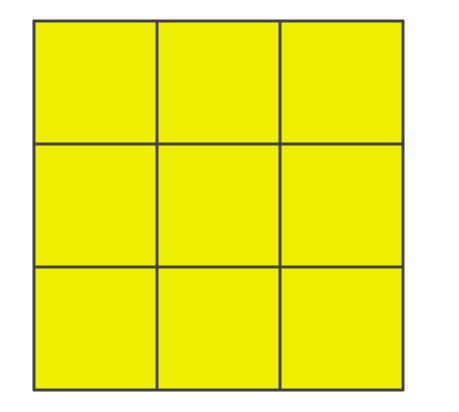Solution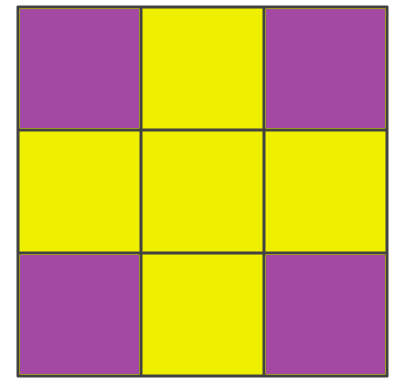∴ Above figure represents the shaded region 4 / 9 of the whole figure

3. In the given figure, if we say that the shaded region is 1/4, then identify the error in it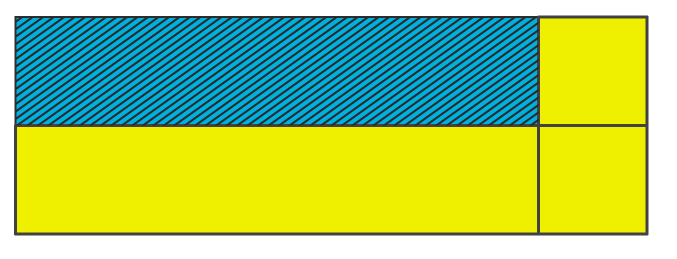Solution

The shaded region is not equal to 1 / 4 since the above figure does not have equal parts

4. Write a fraction for each of the following:

(i) Three- fourths

(ii) Four- sevenths

(iii) Two – fifths

(iv) Three – tenths

(v) One-eighth

(vi) Five- sixths

(vii) Eight- ninths

(viii) Seven-twelfths

Solution

(i) The fraction for three – fourths is 3 / 4

(ii) The fraction for four – sevenths is 4 / 7

(iii)The fraction for two – fifths is 2 / 5

(iv) The fraction for three – tenths is 3 / 10

(v) The fraction for one- eighth is 1 / 8

(vi) The fraction for five – sixths is 5 / 6

(vii) The fraction for eight – ninths is 8 / 9

(viii) The fraction for seven – twelfths is 7 / 12

5. Write down the numerator and the denominator of each of the fractions given below:

(i) 4 / 9 (ii) 6 / 11 (iii) 8 / 15 (iv) 12 / 17 (v) 5 / 1

Solutions

(i) 4 / 9

Numerator of 4 / 9 is 4

Denominator of 4 / 9 is 9

(ii) 6 / 11

Numerator of 6 / 11 is 6

Denominator of 6 / 11 is 11

(iii) 8 / 15

Numerator of 8 / 15 is 8

Denominator of 8 / 15 is 15

(iv) 12 / 17

Numerator of 12 / 17 is 12

Denominator of 12 / 17 is 17

(v) 5 / 1

Numerator of 5 / 1 is 5

Denominator of 5 / 1 is 1

6. Write down the fraction in which

(i) numerator = 3, denominator = 8

(ii) numerator = 5, denominator = 12

(iii) numerator = 7, denominator = 16

(iv) numerator = 8, denominator = 15

Solutions

(i) Fraction of numerator = 3, denominator = 8 is 3 / 8

(ii) Fraction of numerator = 5, denominator = 12 is 5 / 12

(iii) Fraction of numerator = 7, denominator = 16 is 7 / 16

(iv) Fraction of numerator = 8, denominator = 15 is 8 / 15

## Exercise 5B PAGE NO: 85

1. Which of the following are proper fractions?

1 / 2, 3 / 5, 10 / 7, 7 / 4, 2, 15 / 8, 16 / 16, 10 / 11, 23 / 10

Solution

A fraction whose numerator is less than its denominator is called a proper fraction

Here,

1 / 2, 3 / 5 and 10 / 11 are proper fractions

2. Which of the following are improper fractions?

3 / 2, 5 / 6, 9 / 4, 8 / 8, 3, 27 / 16, 23 / 31, 19 / 18, 10 / 13, 26 / 26

Solution

A fraction whose numerator is greater than or equal to its denominator is called an improper fraction

Here,

3 / 2, 9 / 4, 8 / 8, 3, 27 / 16, 19 / 18 and 26 / 26 are improper fractions.

3. Write six improper fractions with denominator 5

Solution

6 / 5, 7 / 5, 8 / 5, 9 / 5, 11 / 5, 12 / 5 are improper fractions with denominator 5

4. Write six improper fractions with numerator 13

Solution

13 / 2, 13 / 3 13 / 4, 13 / 5, 13 / 6, 13 / 7 are improper fractions with numerator 13

## Exercise 5C PAGE NO: 89

1. Write five fractions equivalent to each of the following:

(i) 2 / 3

(ii) 4 / 5

(iii) 5 /8

(iv) 7 / 10

(v) 3 / 7

(vi) 6 / 11

(vii) 7 / 9

(viii) 5 / 12

Solution

(i) 2 / 3

2 / 3 = (2 / 3 × 2 / 2) = (2 / 3 × 3 / 3) = (2 / 3 × 4 / 4) = (2 / 3 × 5 / 5) = (2 / 3 × 6 / 6)

∴ 2 / 3 = 4 / 6 = 6 / 9 = 8 / 12 = 10 / 15 = 12 / 18

The fractions are 4 / 6, 6 / 9, 8 / 12, 10 / 15 and 12 / 18

Hence, the five fractions equivalent to 2 / 3 are 4 / 6, 6 / 9, 8 / 12, 10 / 15 and 12 / 18

(ii) 4 / 5

4 / 5 = (4 / 5 × 2 / 2) = (4 / 5 × 3 / 3) = (4 / 5 × 4 / 4) = (4 / 5 × 5 / 5) = (4 / 5 × 6 / 6)

∴ 4 / 5 = 8 / 10 = 12 / 15 = 16 / 20 = 20 / 25 = 24 / 30

The five fractions are 8 / 10, 12 / 15, 16 / 20, 20 / 25 and 24 / 30

Hence, the five fractions equivalent to 4 / 5 are 8 / 10, 12 / 15, 16 / 20, 20 / 25 and 24 / 30

(iii) 5/8

5 / 8 = (5 / 8 × 2 / 2) = (5 / 8 × 3 / 3) = (5 / 8 × 4 / 4) = (5 / 8 × 5 / 5) = (5 / 8 × 6 / 6)

∴ 5 / 8 = 10 / 16 = 15 / 24 = 20 / 32 = 25 / 40 = 30 / 48

The five fractions are 10 / 16, 15 / 24, 20 / 32, 25 / 40 and 30 / 48

Hence, the five fractions equivalent to 5 / 8 are 10 / 16, 15 / 24, 20 / 32, 25 / 40 and 30 / 48

(iv) 7/10

7/10 = (7/10 × 2 / 2) = (7 / 10 × 3 / 3) = (7 / 10 × 4 / 4) = (7 / 10 × 5 / 5) = (7 / 10 × 6 / 6)

∴ 7 / 10 = 14 / 20 = 21 / 30 = 28 / 40 = 35/ 50 = 42 / 60

The five fractions are 14 / 20, 21 / 30, 28 / 40, 35 / 50 and 42 / 60

Hence, the five fractions equivalent to 7 / 10 are 14 / 20, 21 / 30, 28 / 40, 35 / 50 and 42 / 60

(v) 3 / 7

3 / 7 = (3 / 7 × 2 / 2) = (3 / 7 × 3 / 3) = (3 / 7 × 4 / 4) = (3 / 7 × 5 / 5) = (3 / 7 × 6 / 6)

∴ 3 / 7 = 6 / 14 = 9 / 21 = 12 / 28 = 15 / 35 = 18 / 42

The five fractions are 6 / 14, 9 / 21, 12 / 28, 15 / 35 and 18 / 42

Hence, the five fractions equivalent to 3 / 7 are 6 / 14, 9 / 21, 12 / 28, 15 / 35 and 18 / 42

(vi) 6 / 11

6 / 11 = (6 / 11 × 2 / 2) = (6 / 11 × 3 / 3) = (6 / 11 × 4 / 4) = (6 / 11 × 5 / 5) = (6 / 11 × 6 / 6)

∴6 / 11 = 12 / 22 = 18 / 33 = 24 / 44 = 30 / 55 = 36 / 66

The five fractions are 12 / 22, 18 / 33, 24 / 44, 30 / 55 and 36 / 66

Hence, the five fractions equivalent to 6 / 11 are 12 / 22, 18 / 33, 24 / 44, 30 / 55 and 36 / 66

(vii) 7/ 9

7/ 9 = (7 / 9 × 2 / 2) = (7 / 9 × 3 / 3) = (7 / 9 × 4 / 4) = (7 / 9 × 5 / 5) = (7 / 9 × 6 / 6)

∴ 7 / 9 = 14 / 18 = 21 / 27 = 28 / 36 = 35 / 45 = 42 / 54

The five fractions are 14 / 18, 21 / 27, 28 / 36, 35 / 45 and 42 / 54

Hence, the five fractions equivalent to 7 / 9 are 14 / 18, 21 / 27, 28 / 36, 35 / 45 and 42 / 54

(viii) 5 / 12

5 / 12 = (5 / 12 × 2 / 2) = (5 / 12 × 3 / 3) = (5 / 12 × 4 / 4) = (5 / 12 × 5 / 5) = (5 / 12 × 6 / 6)

∴ 5 / 12 = 10 / 24 = 15 / 36 = 20 / 48 = 25 / 60 = 30 / 72

The five fractions equivalent to 5 / 12 are 10 / 24, 15 / 36, 20 / 48, 25 / 60 and 30 / 72

2. Which of the following are the pairs of equivalent fractions?

(i) 5 / 6 and 20 / 24

(ii) 3 / 8 and 15 / 40

(iii) 4 / 7 and 16 / 21

(iv) 2 / 9 and 14 / 63

(v) 1 / 3 and 9 / 24

(vi) 2 / 3 and 33 / 22

Solutions

(i) 5 / 6 and 20 / 24

Given fractions are 5 / 6 and 20 / 24

By cross multiplication we get

(5 × 24) = 120

(6 × 20) = 120

Now (5 × 24) = (6 × 20) = 120

Hence, 5 / 6 and 20 / 24 are the pairs of equivalent fractions

(ii) 3 / 8 and 15 / 40

Given fractions are 3 / 8 and 15 / 40

By cross multiplication we get

(3 × 40) = 120

(8 × 15) = 120

Now (3 × 40) = (8 × 15) = 120

Hence, 3 / 8 and 15 / 40 are the pairs of equivalent fractions

(iii) 4 / 7 and 16 / 21

Given fractions are 4 / 7 and 16 / 21

By cross multiplication we get

(4 × 21) = 84

(7 × 16) = 112

Now (4 × 21) ≠ (7 × 16)

Hence, 4 / 7 and 16 / 21 are not the pairs of equivalent fractions

(iv) 2 / 9 and 14 / 63

Given fractions are 2 / 9 and 14 / 63

By cross multiplication we get

(2 × 63) = 126

(9 × 14) = 126

Now (2 × 63) = (9 × 14) = 126

Hence, 2 / 9 and 14 / 63 are the pairs of equivalent fractions

(v) 1 / 3 and 9 / 24

Given fractions are 1 / 3 and 9 / 24

By cross multiplication we get

(1 × 24) = 24

(3 × 9) = 27

Now (1 × 24) ≠ (3 × 9)

Hence,1 / 3 and 9 / 24 are not the pairs of equivalent fractions

(vi) 2 / 3 and 33 / 22

Given fractions are 2 / 3 and 33 / 22

By cross multiplication we get

(2 × 22) = 44

(3 × 33) = 99

Now (2 × 22) ≠ (3 × 33)

Hence, 2 / 3 and 33 / 22 are not the pairs of equivalent fractions

3. Find the equivalent fraction of 3 / 5 having

(i) denominator 30 (ii) numerator 24

Solution

(i) Let 3 / 5 = ☐ / 30

Clearly shows 30 = (5 × 6)

Now multiply the numerator by 6 also

∴ 3 / 5 = (3 × 6) / (5 × 6) = 18 /30

Hence, 18 / 30 is the equivalent fraction of 3 / 5 having denominator 30

(ii) Let 3 / 5 = 24 / ☐

Clearly shows 24 = (3 × 8)

Now multiply the denominator by 8 also

∴ 3 / 5 = (3 × 8) / (5 × 8) = 24 / 40

Hence, 24 / 40 is the equivalent fraction of 3 / 5 having numerator 24

4. Find the equivalent fraction of 5 / 9 having

(i) denominator 54 (ii) numerator 35

Solution

(i) Let 5 / 9 = ☐ / 54

Clearly shows 54 = (9 × 6)

Now multiply the numerator by 6 also

∴ 5 / 9 = (5 × 6) / (9 × 6) = 30 / 54

Hence, 30 / 54 is the equivalent fraction of 5 / 9 having denominator 54

(ii) Let 5 / 9 = 35 / ☐

Clearly shows 35 = (5 × 7)

Now multiply the denominator by 7 also

∴ 5 / 9 = (5 × 7) / (9 × 7) = 35 / 63

Hence, 35 / 63 is the equivalent fraction of 5 / 9 having numerator 35

5. Find the equivalent fraction of 6 / 11 having

(i) denominator 77 (ii) numerator 60

Solution

(i) Let 6 / 11 = ☐ / 77

Clearly shows 77 = (11 × 7)

Now multiply the numerator by 7 also

∴ 6 / 11 = (6 × 7) / (11 × 7) = 42 / 77

Hence, 42 / 77 is the equivalent fraction of 6 / 11 having denominator 77

(ii) Let 6 / 11 = 60 / ☐

Clearly shows 60 = (6 × 10)

Now multiply the denominator by 10 also

∴ 6 / 11 = (6 × 10) / (11 × 10) = 60 / 110

Hence, 60 / 110 is the equivalent fraction of 6 / 11 having numerator 60

## Exercise 5D PAGE NO: 93

1. Define like and unlike fractions and give five examples of each.

Solution

Like fractions

Fractions having the same denominator are called ‘Like fractions’

The five examples of like fractions are

2 / 7, 3 / 7, 4 / 7, 5 / 7 and 6 / 7

Unlike fractions

Fractions having different denominators are called ‘Unlike fractions’

The five examples of unlike fractions are

2 / 6, 4 / 7, 5 / 9, 6 / 8, 9 / 6

2. Convert 3 / 5, 7 / 10, 8 / 15 and 11 / 30 into like fractions

Solution

Given fractions are 3 / 5, 7 / 10, 8 / 15 and 11 / 30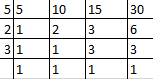LCM of 5, 10, 15 and 30 = (5 × 3 × 2) = 30

Converting each of the given fractions into an equivalent fraction with denominator as 30

We get

(3 × 6) / (5 × 6) = 18 / 30

(7 × 3) / (10 × 3) = 21 / 30

(8 × 2) / (15 × 2) = 16 / 30

(11 × 1) / (30 × 1) = 11 / 30

∴ 18 / 30, 21 / 30, 16 / 30 and 11 / 30 are the required like fractions

3. Convert 1 / 4, 5 / 8, 7 / 12 and 13 / 24 into like fractions

Solution

Given fractions are 1 / 4, 5 / 8, 7 / 12 and 13 / 24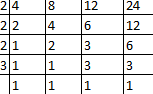LCM of 4, 8, 12, 24 = (2 × 2 × 2 × 3) = 24

Converting each of the given fractions into an equivalent fraction with denominator as 24

We get

(1 × 6) / (4 × 6) = 6 / 24

(5 × 3) / (8 × 3) = 15 / 24

(7 × 2) / (12 × 2) = 14 / 24

(13 × 1) / (24 × 1) = 13 / 24

∴ 6 / 24, 15 / 24, 14 / 24 and 13 / 24 are the required like fractions

4. Fill in the place holders with the correct symbol > or <:

(i) 8 / 9 ☐ 5 / 9

(ii) 9 / 10 ☐ 7 / 10

(iii) 3 / 7 ☐ 6 / 7

(iv) 11 / 15 ☐ 8 / 15

(v) 6 / 11 ☐ 5 / 11

(vi) 11 / 20 ☐ 17 / 20

Solutions

(i) Since among the two fractions with the same denominator, the one with the greater

numerator is the greater of the two

Hence, 8 / 9 > 5 / 9

(ii) Since among the two fractions with the same denominator, the one with the greater

numerator is the greater of the two

Hence, 9 / 10 > 7 / 10

(iii) Since among the two fractions with the same denominator, the one with the greater

numerator is the greater of the two

Hence, 3 / 7 < 6 / 7

(iv)Since among the two fractions with the same denominator, the one with the greater

numerator is the greater of the two

Hence, 11 / 15 > 8 / 15

(v) Since among the two fractions with the same denominator, the one with the greater

numerator is the greater of the two

Hence, 6 / 11 > 5 / 11

(vi)Since among the two fractions with the same denominator, the one with the greater

numerator is the greater of the two

Hence, 11 / 20 < 17 / 20

5. Fill in the place holders with the correct symbol > or <:

(i) 3 / 4 ☐ 3 / 5

(ii) 7 / 8 ☐ 7 / 10

(iii) 4 / 11 ☐ 4 / 9

(iv) 8 / 11 ☐ 8 / 13

(v) 5 / 12 ☐ 5 / 8

(vi) 11 / 4 ☐ 11 / 15

Solutions

(i) Since among two fractions with same numerator, the one with the smaller denominator is the

greater of the two

Hence 3 / 4 > 3 / 5

(ii) Since among two fractions with same numerator, the one with the smaller denominator is the

greater of the two

Hence, 7 / 8 > 7 / 10

(iii) Since among two fractions with same numerator, the one with the smaller denominator is the

greater of the two

Hence, 4 / 11 < 4 / 9

(iv) Since among two fractions with same numerator, the one with the smaller denominator is the

greater of the two

Hence, 8 / 11 > 8 / 13

(v) Since among two fractions with same numerator, the one with the smaller denominator is the

greater of the two

Hence, 5 / 12 < 5 / 8

(vi) Since among two fractions with same numerator, the one with the smaller denominator is the

greater of the two

Hence, 11 / 4 > 11 / 15

Compare the fractions given below:

6. 4 / 5, 5 / 7

Solution

Given fractions are 4 / 5 and 5 / 7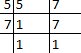LCM of 5 and 7 = (5 × 7) = 35

Now convert each one of 4 / 5 and 5 / 7 into an equivalent fraction having 35 as denominator

4 / 5 = (4 × 7) / (5 × 7) = 28 / 35

5 / 7 = (5 × 5) / (7× 5) = 25 / 35

Clearly it shows 28 / 35 > 25 / 35

Hence, 4 / 5 > 5 / 7

7. 3 / 8, 5 / 6

Solution

Given fractions are 3 / 8 and 5 / 6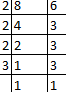LCM of 8 and 6 = (2 × 2 × 2 × 3) = 24

Now convert each one of 3 / 8 and 5 / 6 into an equivalent fraction having 24 as denominator

3 / 8 = (3 × 3) / (8 × 3) = 9 / 24

5 / 6 = (5 × 4) / (6 × 4) = 20 / 24

Clearly it shows 9 / 24 < 20 / 24

Hence, 3 / 8 < 5 / 6

8. 7 / 11, 6 / 7

Solution

Given fractions are 7 / 11 and 6 / 7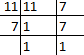LCM of 11 and 7 = (11 × 7) = 77

Now convert each one of 7/11 and 6/7 into an equivalent fraction having 77 as denominator

7 / 11 = (7 × 7) / (11 × 7) = 49 / 77

6 / 7 = (6 × 11) / (7 × 11) = 66 / 77

Clearly it shows 49 / 77 < 66 / 77

Hence, 7 / 11 < 6 / 7

9. 5 / 6, 9 / 11

Solution

Given fractions are 5 / 6 and 9 / 11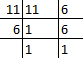LCM of 11 and 6 = (11 × 6) = 66

Now convert each one of 5 / 6 and 9 / 11 into an equivalent fraction having 66 as

denominator

5 / 6 = (5 × 11) / (6 × 11) = 55 / 66

9 / 11 = (9 × 6) / (11 × 6) = 54 / 66

Clearly it shows 55 / 66 > 54 / 66

Hence, 5 / 6 > 9 / 11

10. 2 / 3, 4 / 9

Solution

Given fractions are 2 / 3 and 4 / 9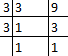LCM of 3 and 9 = (3 × 3) = 9

Now convert each one of 2 / 3 and 4 / 9 into an equivalent fraction having 9 as

denominator

2 / 3 = (2 × 3) / (3 × 3) = 6 / 9

4 / 9 = (4 ×1) / (9 × 1) = 4 / 9

Clearly it shows 6 / 9 > 4 / 9

Hence, 2 / 3 > 4 / 9

11. 6 / 13, 3 / 4

Solution

Given fractions are 6 / 13 and 3 / 4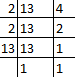LCM of 13 and 4 = (2 × 2 × 13) = 52

Now convert each one of 6/13 and 3/4 into an equivalent fraction having 52 as denominator

6 / 13 = (6 × 4) / (13 × 4) = 24 / 52

3 / 4 = (3 × 13) / (4 × 13) = 39 / 52

Clearly it shows 24/52 < 39/52

Hence, 6 / 13 < 3 / 4

12. 3 / 4, 5 / 6

Solution

Given fractions are 3 / 4 and 5 / 6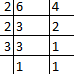LCM of 4 and 6 = (2 × 2 × 3) = 12

Now convert each of 3 / 4 and 5 / 6 into an equivalent fraction having 12 as

denominator

3 / 4 = (3 × 3) / (4× 3) = 9 / 12

5 / 6 = (5 × 2) / (6 × 2) = 10 / 12

Clearly it shows 9 / 12 < 10 / 12

Hence, 3 / 4 < 5 / 6

13. 5 / 8, 7 / 12

Solution

Given fractions are 5 / 8 and 7 / 12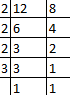LCM of 8 and 12 = 24

Now convert each of 5 / 8 and 7 / 12 into an equivalent fraction having 24 as

denominator

5 / 8 = (5 × 3) / (8 × 3) = 15 / 24

7 / 12 = (7 × 2) / (12 × 2) = 14 / 24

Clearly it shows 15 / 24 > 14 / 24

Hence, 5 / 8 > 7 / 12

14. 4 / 9, 5 / 6

Solution

Given fractions are 4 / 9 and 5 / 6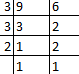LCM of 9 and 6 = (3 × 3 × 2) = 18

Now convert each of 4 / 9 and 5 / 6 into an equivalent fraction having 18 as

denominator

4 / 9 = (4 × 2) / (9 × 2) = 8 / 18

5 / 6 = (5 × 3) / (6 × 3) = 15 / 18

Clearly it shows 8 / 18 < 15 / 18

Hence, 4 / 9 < 5 / 6

15. 4 / 5, 7 / 10

Solution

Given fractions are 4 / 5 and 7 / 10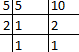LCM of 5 and 10 = (5 × 2) = 10

Now convert each of 4 / 5 and 7 / 10 into an equivalent fraction having 10 as

denominator

4 / 5 = (4 × 2) / (5 × 2) = 8 / 10

7 / 10 = (7× 1) / (10 ×1) = 7 / 10

Clearly it shows 8 / 10 > 7 / 10

Hence, 4 / 5 > 7 / 10

## Exercise 5E PAGE NO: 96

Find the sum:

1. 5 / 8 + 1 / 8

Solution

Given 5 / 8 + 1 / 8

5 / 8 + 1 / 8 = (5 + 1) / 8

= 6 / 8

= 3 / 4

∴ Sum of 5 / 8 + 1 / 8 = 3 / 4

2. 4 / 9 + 8 / 9

Solution

Given 4 / 9 + 8 / 9

4 / 9 + 8 / 9 = (4 + 8) / 9

= 12 / 9

= 4 / 3

∴ Sum of 4 / 9 + 8 / 9 = 4 / 3

=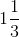3.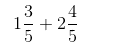Solution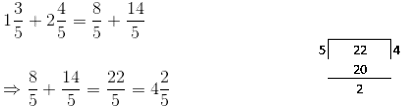4. 2 / 9 + 5 / 6

Solution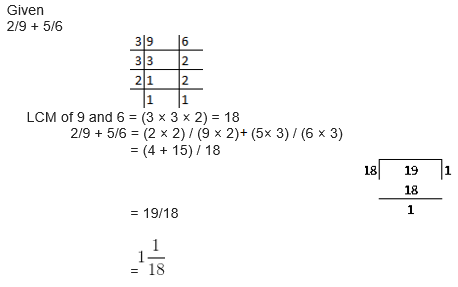5. 7 / 12 + 9 / 16

Solution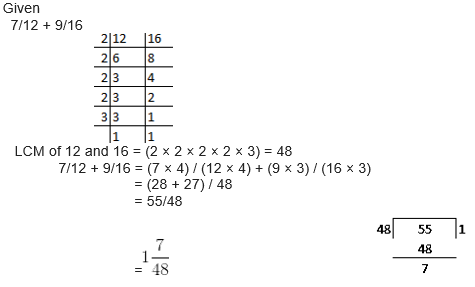6. 4 / 15 + 17 / 20

Solution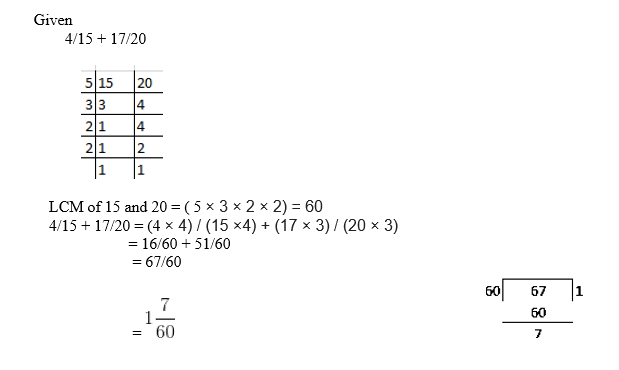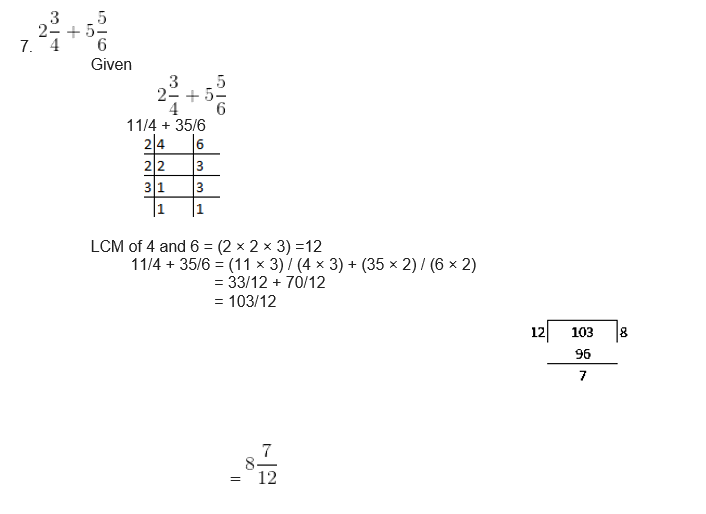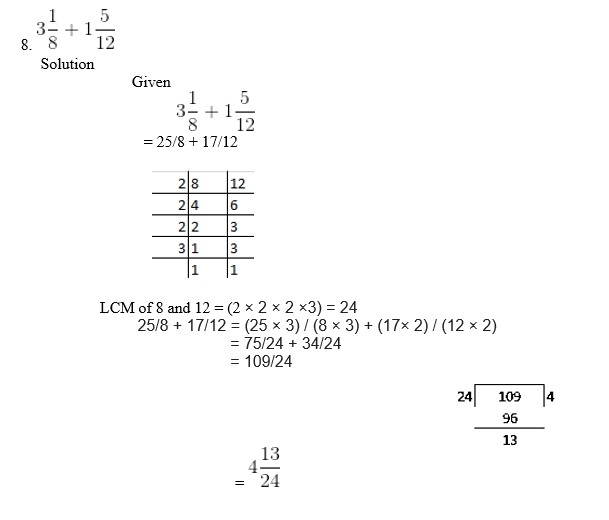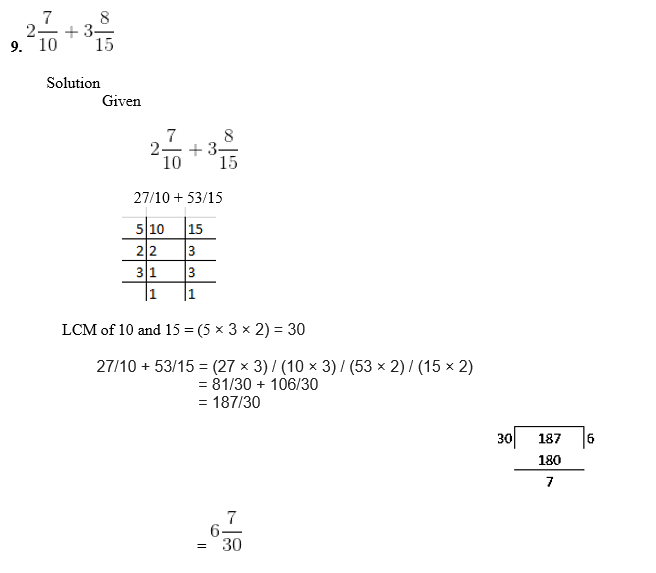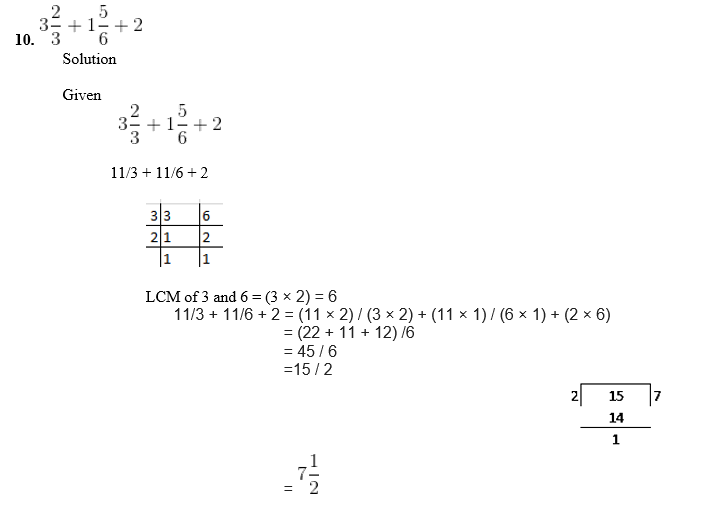## Exercise 5F PAGE NO: 99

Find the difference:

1. 5 / 8 – 1 / 8

Solution

We have

5 / 8 – 1 / 8

5 / 8 – 1 / 8 = (5-1) / 8

= 4 / 8

= 2 / 4

= 1 / 2

Hence, 5 / 8 – 1 / 8 = 1 / 2

2. 7 / 12 – 5 / 12

Solution

We have

7 / 12 – 5 / 12

7 / 12 – 5 / 12 = (7 – 5) / 12

= 2 / 12

= 1 / 6

Hence, 7 /12 – 5 / 12 = 1 / 6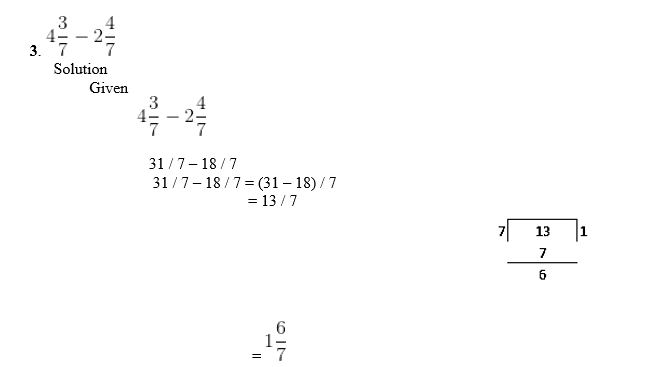4. 5 / 6 – 4 / 9

Solution

Given

5 / 6 – 4 / 9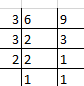LCM of 6 and 9 = (3 × 3 × 2) = 18

Now, 5 / 6 = (5 × 3) / (6 × 3) = 15 / 18

4 / 9 = (4 × 2) / (9 × 2) = 8 / 18

∴ 5 / 6 – 4 / 9 = 15 / 18 – 8 /18

= (15 – 8) / 18

= 7 / 18

Hence, 5 / 6 – 4 / 9 = 7 / 18

5. 1 / 2 – 3 / 8

Solution

Given

1 / 2 – 3 / 8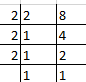LCM of 2 and 8 = (2 × 2 × 2) = 8

Now, 1 / 2 = (1 × 4) / (2 ×4) = 4 / 8

3 / 8 = (3 × 1) / (8 × 1) = 3 / 8

∴ 1 / 2 – 3 / 8 = 4 /8 -3 / 8

= (4 – 3) / 8

= 1 / 8

Hence, 1 / 2 – 3 / 8 = 1 / 8

6. 5 / 8 – 7 / 12

Solution

Given

5 / 8 – 7 / 12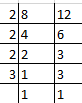LCM of 8 and 12 = (2 × 2 × 2 ×3) = 24

Now, 5 / 8 = (5 × 3) / (8 × 3) = 15 / 24

7 / 12 = (7 × 2) / (12 × 2) = 14 / 24

∴5 / 8 – 7 / 12 = 15 / 24 – 14 / 24

= (15 – 14) / 24

= 1 / 24

Hence, 5 / 8 – 7 / 12 = 1 / 24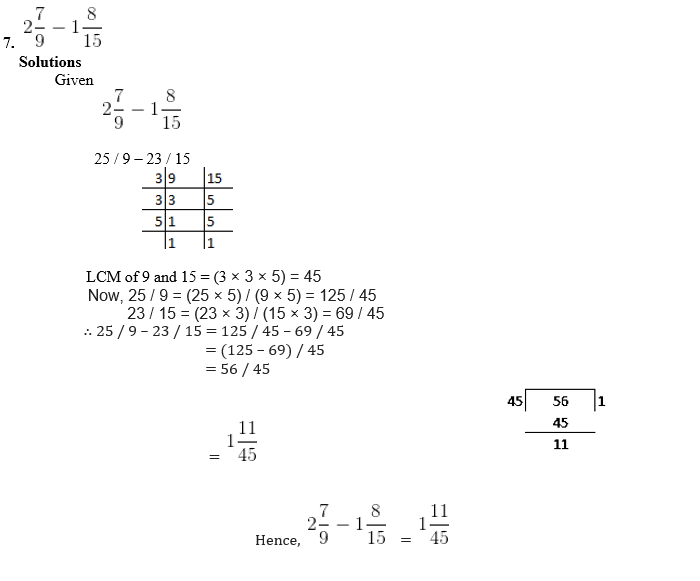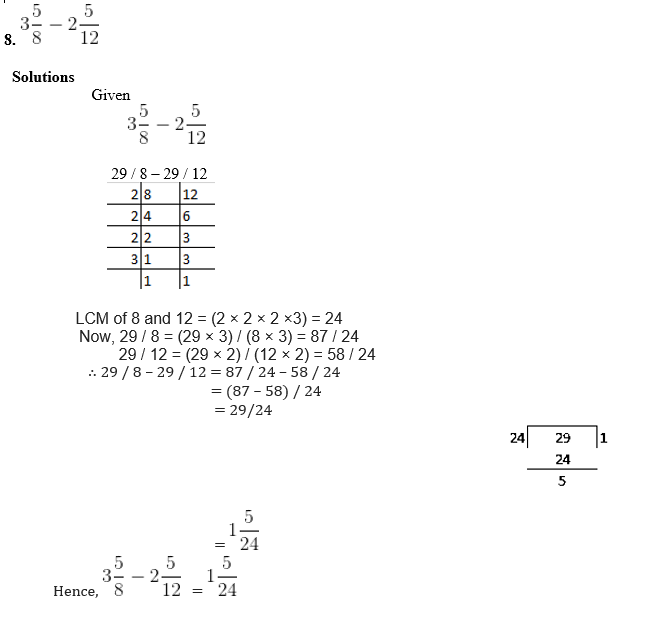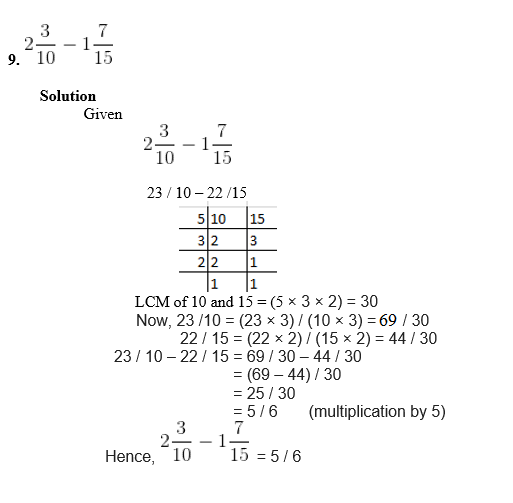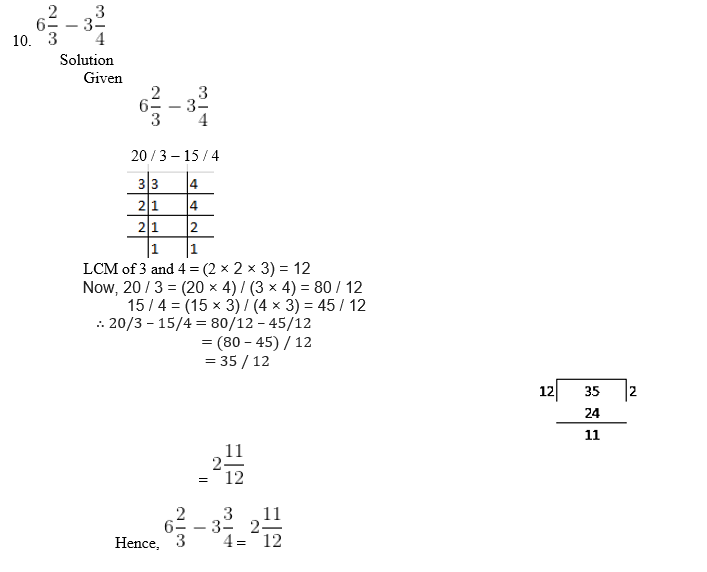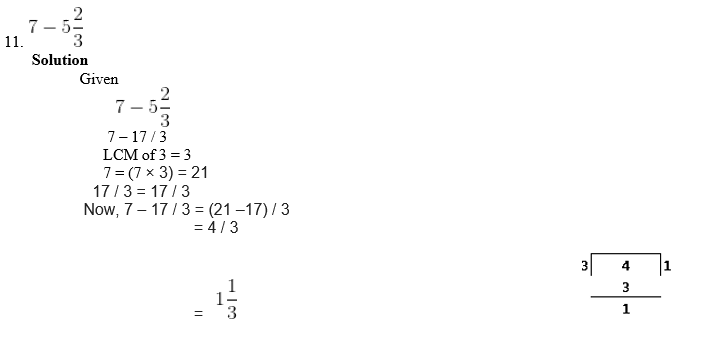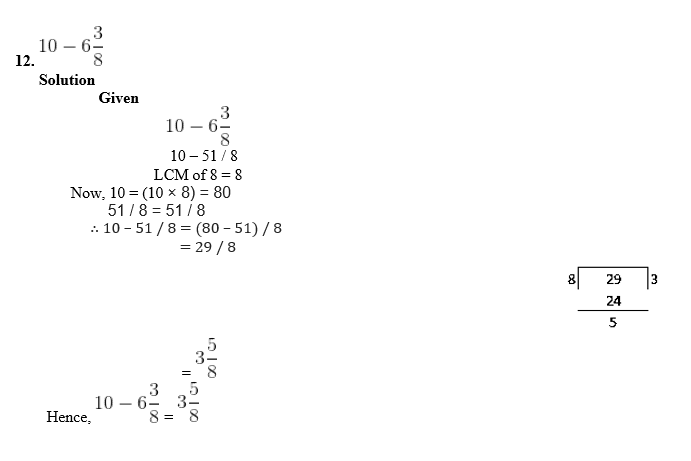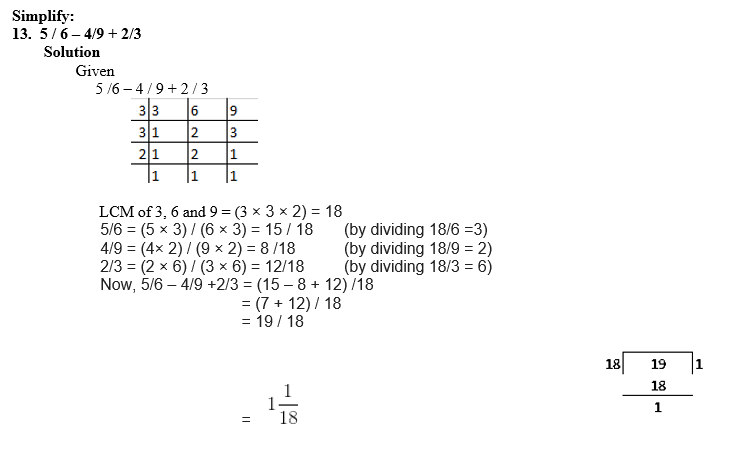14. 5 / 8 + 3 / 4 – 7 / 12

Solution

Given

5 / 8 + 3 /4 – 7 / 12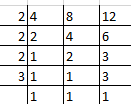LCM of 4, 8 and 12 = (2 × 2 × 2 × 3) = 24

5 / 8 = (5 × 3) / (8 ×3) = 15 / 24 (by dividing 24 / 8 =3)

3 / 4 = (3 × 6) / (4 × 6) = 18 / 24 (by dividing 24 / 4 = 6)

7 / 12 = (7 × 2) / (12 × 2) = 14 / 24 (by dividing 24 / 12 = 2)

Now, 5 / 8 + 3 / 4 – 7 / 12 = (15 + 18 – 14) / 24

= (33 – 14) / 24

= 19 / 24

Hence, 5 / 8 + 3 / 4 – 7 / 12 = 19 / 24

15. 2 + 11 / 15 – 5 / 9

Solution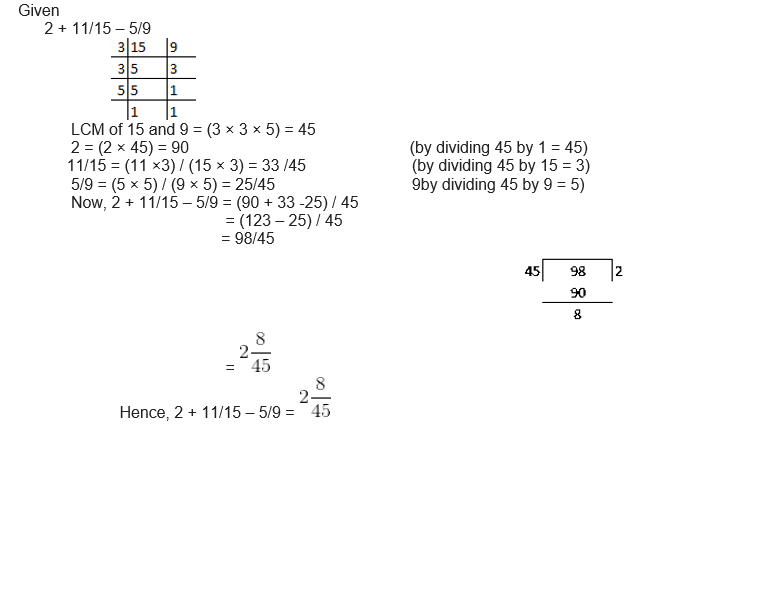## Exercise 5G PAGE NO: 99

1. A fraction equivalent to 3 / 5 is

(a) 3 + 2 / 5 + 2 (b) 3 – 2 / 5 – 2 (c) 3 × 2 / 5 × 2 (d) none of these

Solution

Since two or more fractions representing the same part of a whole are called equivalent fraction

Thus 3 × 2 / 5 × 2 is equivalent fraction to 3 / 5

2. A fraction equivalent to 8 / 12 is

(a) 8 + 4 / 12 + 4 (b) 8 – 4 / 12 – 4 (c) 8 ÷ 4 / 12 ÷ 4 (d) none of these

Solution

Since two or more fractions representing the same part of a whole are called equivalent fraction

Thus 8 ÷ 4 / 12 ÷ 4 is equivalent fraction to 8 / 12

3. A fraction equivalent to 24 / 36 is

(a) 3 / 4 (b) 2 / 3 (c) 8 / 9 (d) none of these

Solution

1, 2, 3, 4, 6, 8, 12, 24 are the factors of 24

1, 2, 3, 4, 6, 9, 12, 18, 36 are the factors of 36

Common factors of 24 and 36 are 1, 2, 3, 4, 6 and12

HCF = 12

Now dividing both numerator and denominator by 12

= 24 ÷ 12/36 ÷ 12

= 2 / 3

Thus 2 / 3 is the equivalent factor to 24 / 36

4. If 3 / 4 is equivalent to x / 20 then the value of x is

(a) 15 (b) 18 (c) 12 (d) none of these

Solution

Since 3 / 4 = x / 20

20 × 3 = 4x

4x = 60

x = 60 / 4

x = 30 / 2

x = 15

Hence, the value of x = 15

Option (a) is the correct answer

5. If 45 / 60 is equivalent to 3 / x then the value of x is

(a) 4 (b) 5 (c) 6 (d) 20

Solution

Since 45 / 60 = 3 / x

45x = 60 × 3

45x = 180

x = 180 / 45

x = 36 / 9

x = 4

Hence, the value of x = 4

Option (a) is the correct answer

6. Which of the following are like fractions?

(a) 2 / 5, 2 / 7, 2 / 9, 2 / 11 (b) 2 / 3, 3 / 4, 4 / 5, 5 / 6

(c) 1 / 8, 3 / 8, 5 / 8, 7 / 8 (d) none of these

Solution

Fractions having the same denominator are called like fractions

Hence 1 / 8, 3 / 8, 5 / 8 and 7 / 8 are like fractions

Option (c) is the correct answer

7. Which of the following is a proper fraction?

(a) 5 / 3 (b) 5 (c)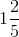(d) none of these

Solution

If the numerator is less than the denominator then the fraction is called as proper fraction

Hence none of these are proper fractions

8. Which of the following is a proper fraction?

(a) 7 / 8 (b)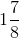(c) 8 / 7 (d) none of these

Solution

If the numerator is less than the denominator then the fraction is called as proper fraction

Hence, 7 / 8 is a proper fraction

9. Which of the following statements is correct?

(a) 3 / 4 < 3 / 5 (b) 3 / 4 > 3 / 5 (c) 3 / 4 and 3 / 5 cannot be compared

Solution

Between the two fractions having the same numerator, the one with the smaller denominator is the greater factor

Hence, 3 / 4 > 3 / 5

Option (b) is the correct answer

10. The smallest of the fractions 3 / 5, 2 / 3, 5 / 6, 7 / 10 is

(a) 2 / 3 (b) 7 / 10 (c) 3 / 5 (d) 5 / 6

Solutions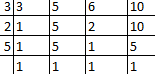LCM of 5, 3, 6 and 10 = (2 × 3 × 5) = 30

Now, 2 / 3 = (2 × 10) / (3 × 10) = 20 / 30 (by dividing 30 / 3 = 10)

7/10 = (7 × 3) / (10 × 3) = 21 / 30 (by dividing 30 / 10 = 3)

3 / 5 = (3 × 6) / (5 × 6) = 18 / 30 (by dividing 30 / 5 = 6)

5 / 6 = (5 × 5) / (6 × 5) = 25/30 (by dividing 30 / 6 = 5)

∴ 18 / 30 is the smallest fraction

Hence, 3 / 5 is the smallest fraction

Option (c) is the correct answer

### RS Aggarwal Solutions for Class 6 Maths Chapter 5 Fractions

Chapter 5 – Fractions consists of 7 exercises. RS Aggarwal Solutions have been solved in detail for each question in every exercise. Let’s have a look at the topics which are included in this Chapter

• Fractions and Fractional Numbers
• Proper, Improper and Mixed Fractions
• Equivalent Fractions
• Like and Unlike Fractions